• 图像的矩阵表示 类和图像类型 虽然使用的是整数坐标， 但 MATLAB 中的像素值（亮度）并未限制为整数。...uint8 和 logical 类广泛用于图像处理， 当以 TIFF 或 JPEG 图像文件格式读取图像时，会用到这两个类
图像的矩阵表示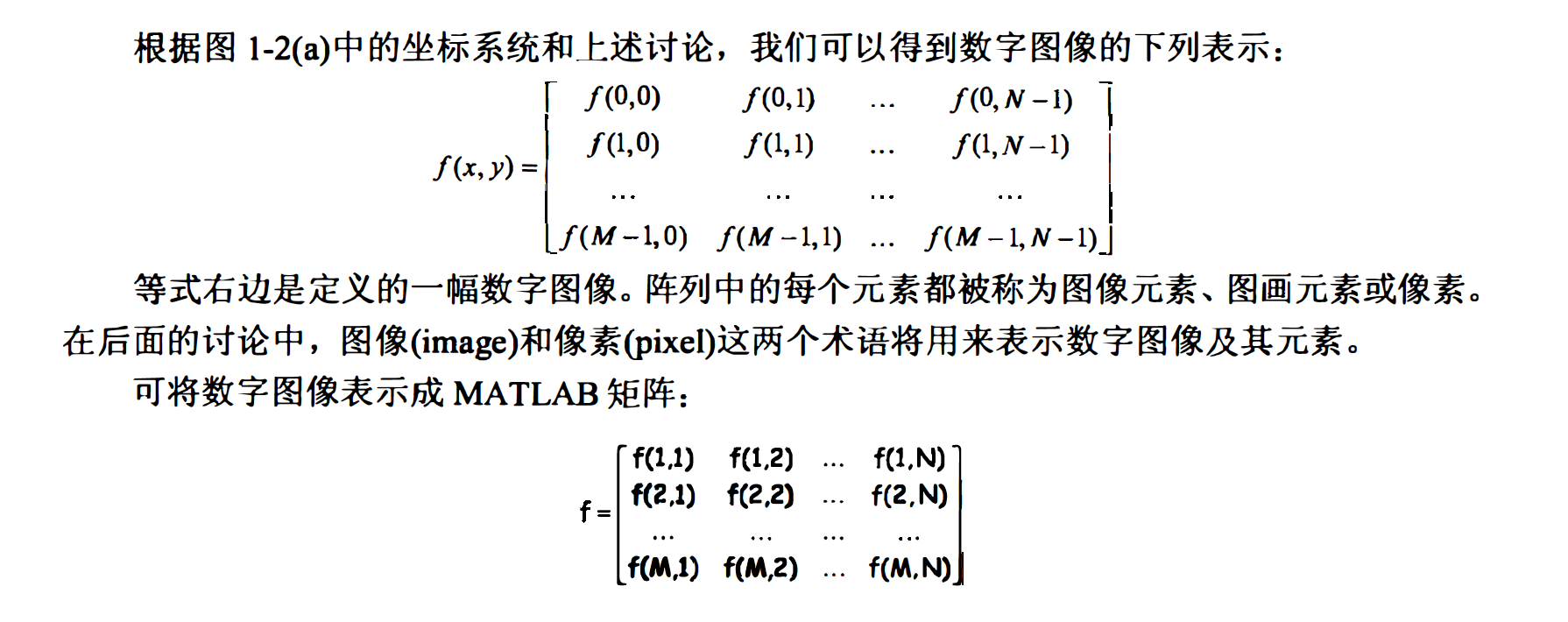类和图像类型
虽然使用的是整数坐标， 但 MATLAB 中的像素值（亮度）并未限制为整数。 表 1-1 列出了 MATLAB 和图像处理工具箱为描述像素值而支持的各种类。 表中的前 8 项是数值型的数据类，第 9 项称为字符类， 最后一项称为逻辑类。
uint8 和 logical 类广泛用于图像处理， 当以 TIFF 或 JPEG 图像文件格式读取图像时，会用到这两个类。 这两个类用1个字节表示每个像素。某些科研数据源， 比如医学成像， 要求提供超出 uint8 的动态范围：针对此类数据， 会采用 uint16 和 int16 类。 这两个类为每个矩阵元素使用2 个字节。针对计算灰度的操作， 比如傅立叶变换（见第 3 章）， 使用 double 和single 浮点类。 双精度浮点数每个数组元素使用8 个宇节， 而单精度浮点数使用 4 个字节。尽管工具箱支持 int8 、 uint32 和 int32 类， 但在图像处理中并不常用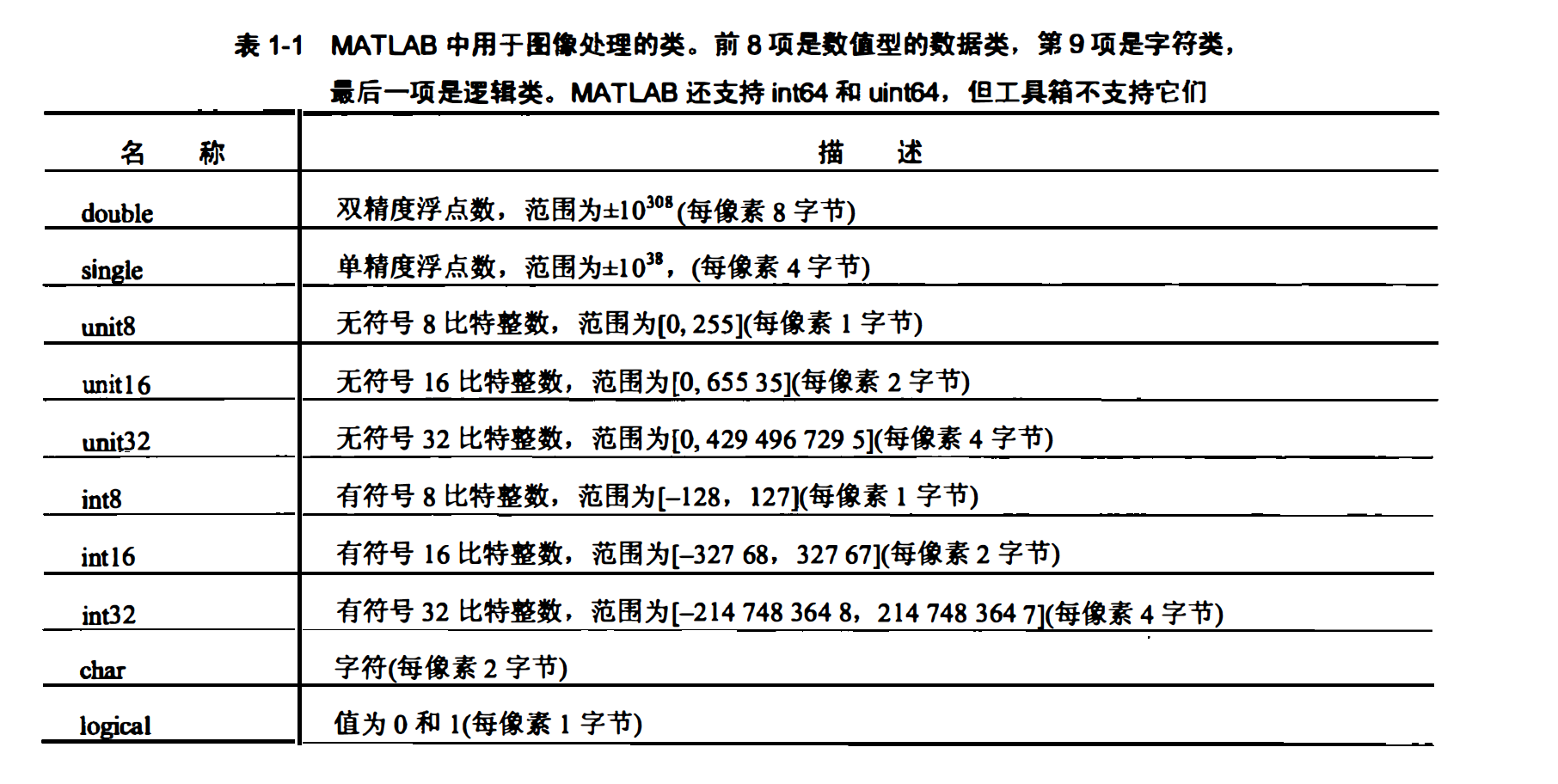算子

一般我们用字母M和N分别表示矩阵中的行与列。1x N
矩阵被称为行向量， M*1矩阵被称为列向量， 1*1矩阵则被称为标量。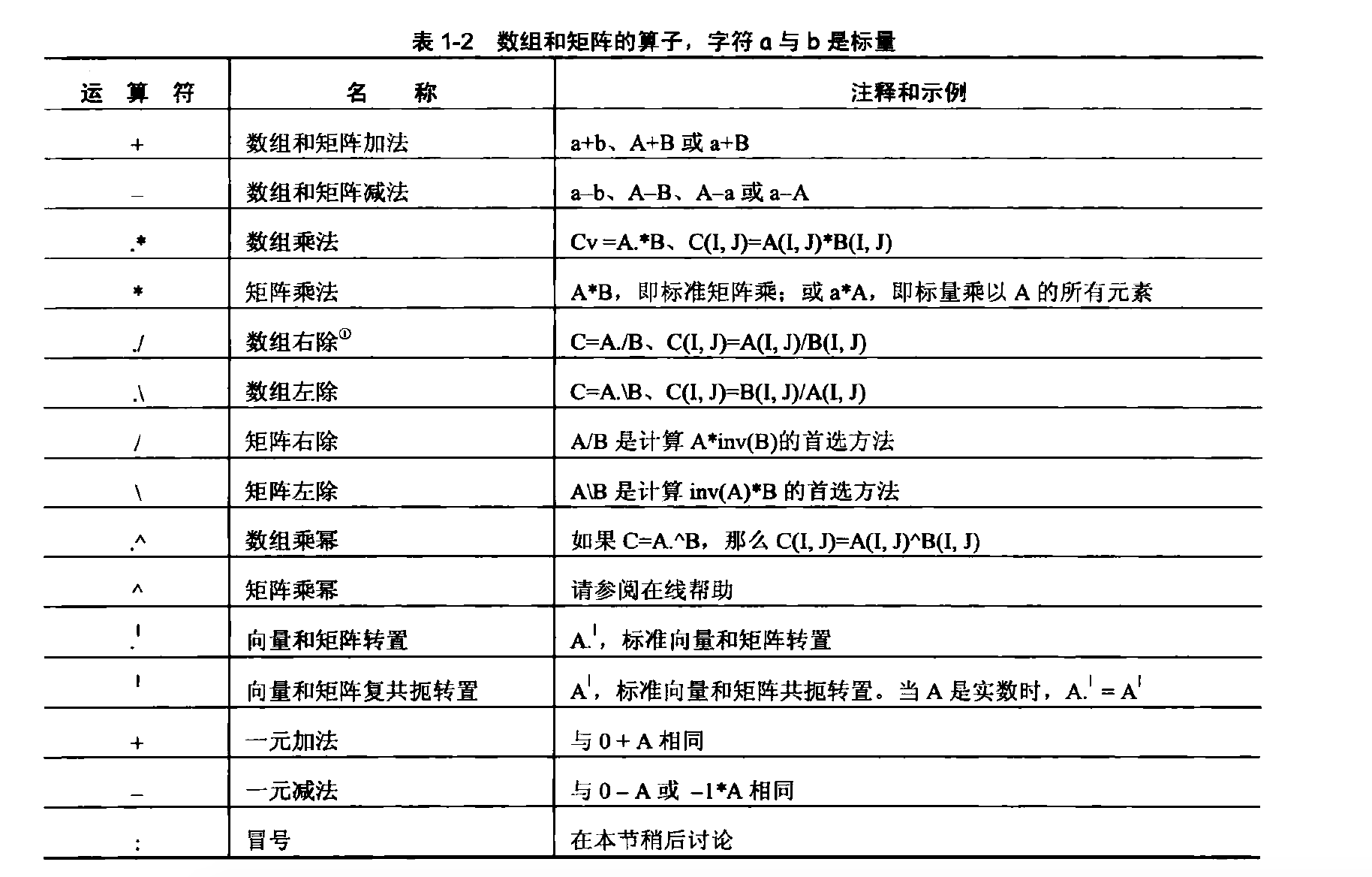Matlab 图像类型及其存储方式

在介绍数字图像的分类时， 曾提及一些主要的图像类型。 本节将介绍这些主 要的图像类型在Matlab中是如何存储和表示的， 主要包括亮度图像、 RGB 图像、 索引图像、二值图像和多帧图像。

1、亮度图像（Intensity Image)
亮度图像即灰度图像。 Matlab使用二维矩阵存储亮度图像，矩阵中的每个元素直接表示 一个像素的亮度〈灰度〉信息。 例如， 一个 200 像素× 300 像素的图像被存储为一个 200 行 300 列的矩阵，可以使用 1.1.5小节介绍的选取矩阵元素〈或子块〉的方式来选择图像中的一个像素或一个区域．

如果矩阵元素的类型是双精度的， 则元素的取值范围是从 0 到 1 ：如果是 8 位无符号整数，则取值范围从0到255。数据0表示黑色，而1（或255）表示最大亮度〈通常为白色〉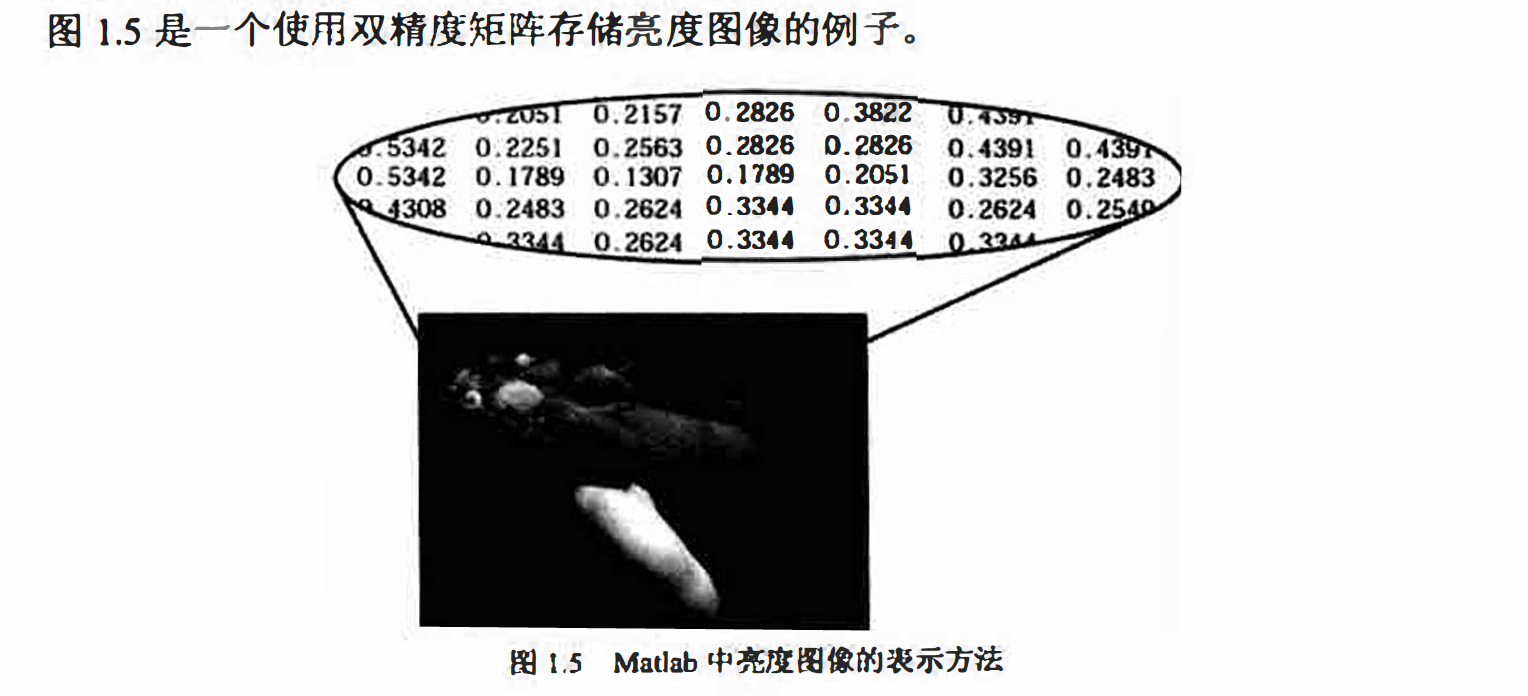2、RGB 图像（RGBlmage)
RGB 图像使用3个一组的数据表达每个像素的颜色， 即其中的红色、绿色和蓝色分量。
在Matlab 中， RGB 图像被存储在一个m ×n×3的三维数组中。对于图像中的每个像素， 存
储的三个颜色分量合成像素的最终颜色。例如， RGB 图像I 中位置在11 行40 列的像素的
RGB 值为I( 11,40.1:3 ）。或I( 11,40,:)，该像素的红色分量为I(11,40,1)，蓝色分量为I( 11,40,3 ）。
而I (:,:,1 ）则表示整个的红色分量图像．

RGB 图像同样可以由观精度数组或8 位无符号整数数组存储。图1.6 是一个使用双精度
数组存储ROB 图像的例子。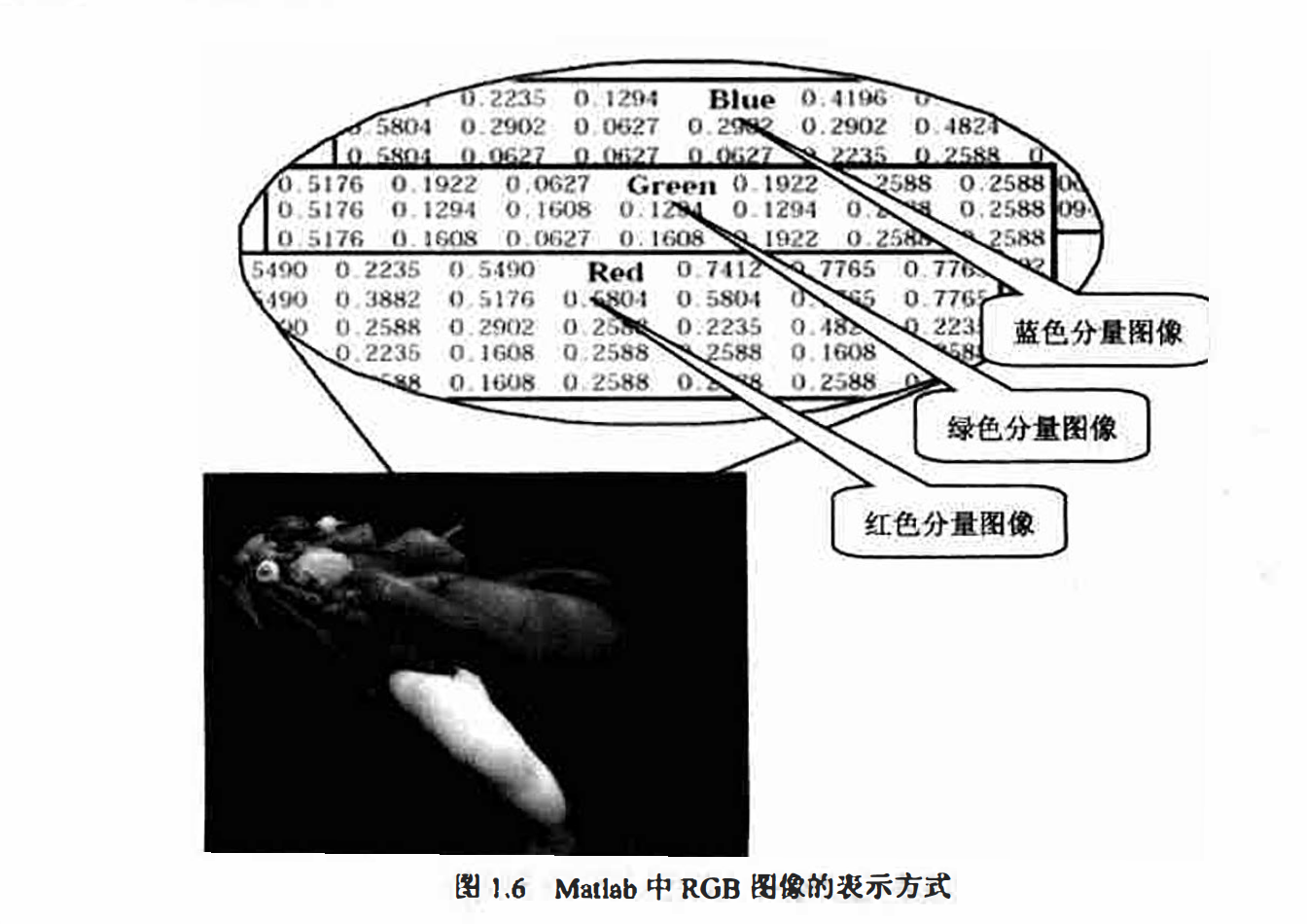3、索引图像（indexed Image)
索引图像往往包含两个数组，一个图像数据矩阵（Image Matrix ）和一个颜色索引表( Colormap ）。对应于图像中的每一个像素， 图像数据数组都包含一个指向颜色索引表的索 引值。
颜色索引表是一个m× 3 的双精度型矩阵， 每一行指定一种颜色的 3 个 RGB 分量，即color = [R G B］。 其中 R、G、 B 是实数类型的现精度数， 取值0～ 1。 0 表示全黑， 1 表示最大亮度。 图 1.7 是一个索引图像的实例， 需要注意的是， 图像中的每个像素都用整数表示， 其含义为颜色索引表中对应颜色的索引。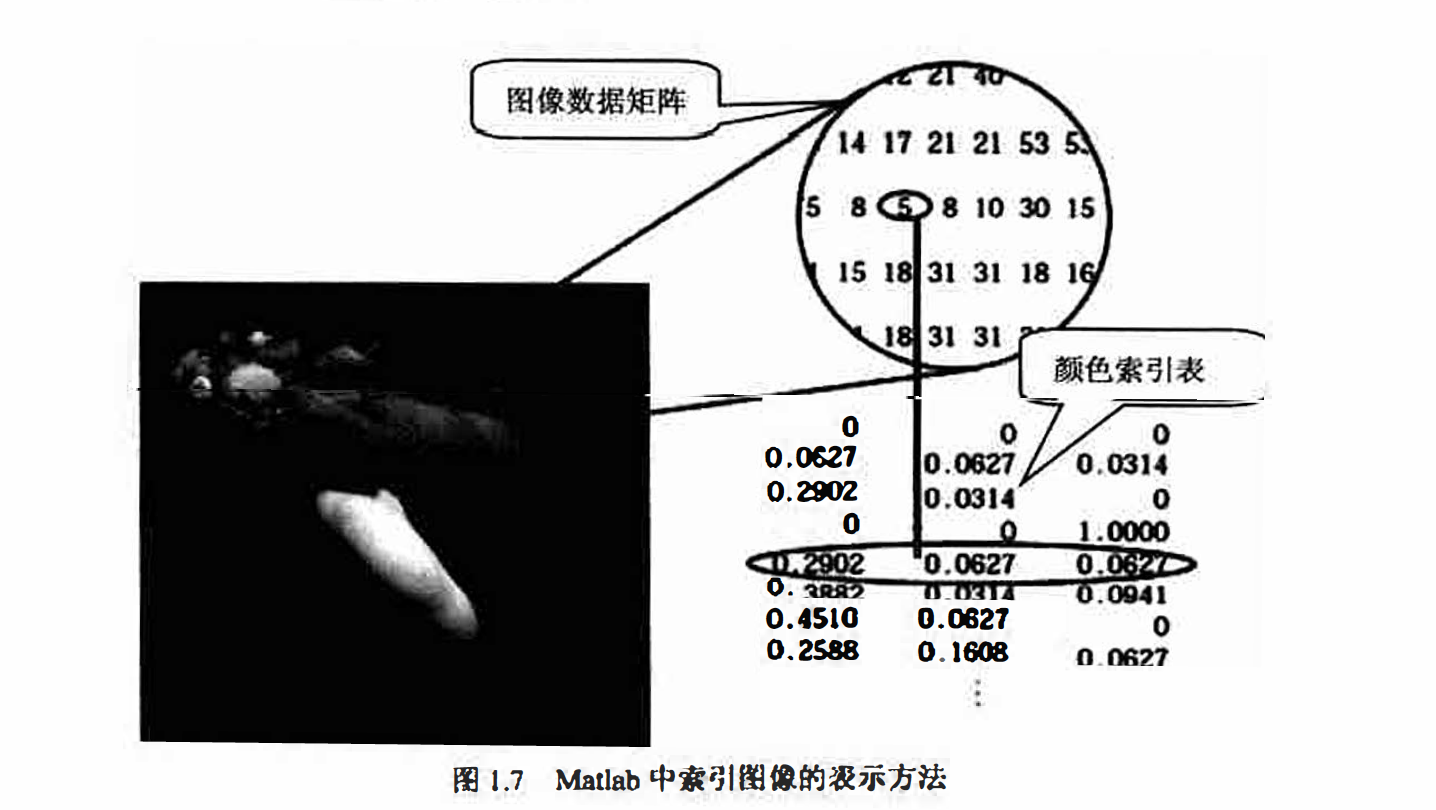图像数据矩阵和颜色索引表的关系取决于图像数据炬阵中存储的数据类型是双精度类
型还是8位无符号整数。
如果图像数据使用双精度类型存储， 则像索数据1表示颜色索引表中的第一行， 像素数
据2表示颜色索引表中的第二行， 以此类推。如果图像数据使用8位无符号整数存储， 则存
在一个额外的偏移量－1， 像素数据0表示颜色索引表中的第一行， 而1表示索引表中的第二
行， 以此类推。
8 位方式存储的图像可以支持256 种颜色（或256 级灰度〉。因1.7 中， 数据矩阵使用的
是双精度类型， 所以没有偏移量． 数据5表示颜色表中的第5种颜色。

4、二值图像（ Binary Image)
在二值图像中， 像素的颜色只有两种取值： 黑或白。Matlab 将二值图像存储为一个二维
矩阵， 每个元素的取值只有0和1两种情况，0表示黑色， 而1表示白色。
二值图像可以被看作是一种特殊的只存在黑和白两种颜色的亮度图像， 当然， 也可以将
二值图像看作是颜色索引表中只存在两种颜色〈黑和白〉的索引图像。

Matlab 中使用uint8 型的逻辑数组存储二值图像， 通过一个逻辑标志表示数据有效范围是0到1，而如果逻辑标志未被置位，则有效范围为0到255。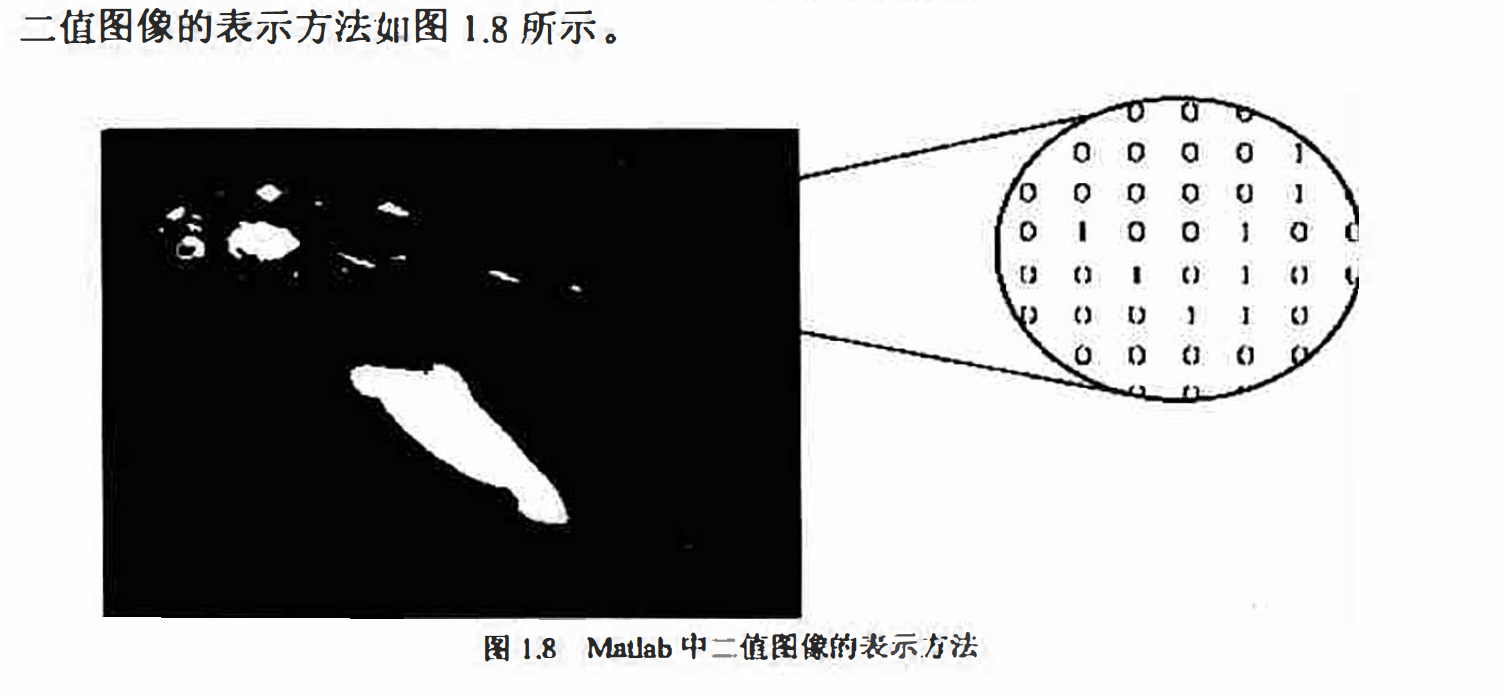5､多帧图像（ Multlframe Image Array)
实际应用中， 可能需要处理多幅按时间或视角方式连续排列的图像， 我们把这种图像称
之为多帧图像（所谓“ 帧” 就是影像动画中最小单位的单幅影像，画面〉。例如核磁共振成像数
据或视频片断. Matlab提供了在同一个矩阵中存储多帧图像的方法， 实际上就是在图像矩阵
中增加一个维度来代表时间或视角信息． 例如， 一个拥有5 张连续的400 像素× 300 像素的
RGB图像的多帧连续片断的存储方式是一个400× 300 × 3× 5 的矩阵， 一组同样大小的灰皮
图像则可以使用一个400× 300 × 1× 5 的矩阵来存储．

如果多帧图像使用索引图像的方式存储，则只有图像数据矩阵被按多帧形式存储， 而颜
色索引表只能公用。因此， 在多帧索引图像中， 所有的索引图像公用一个颜色索引表， 进而
只能使用相同的颜色组合。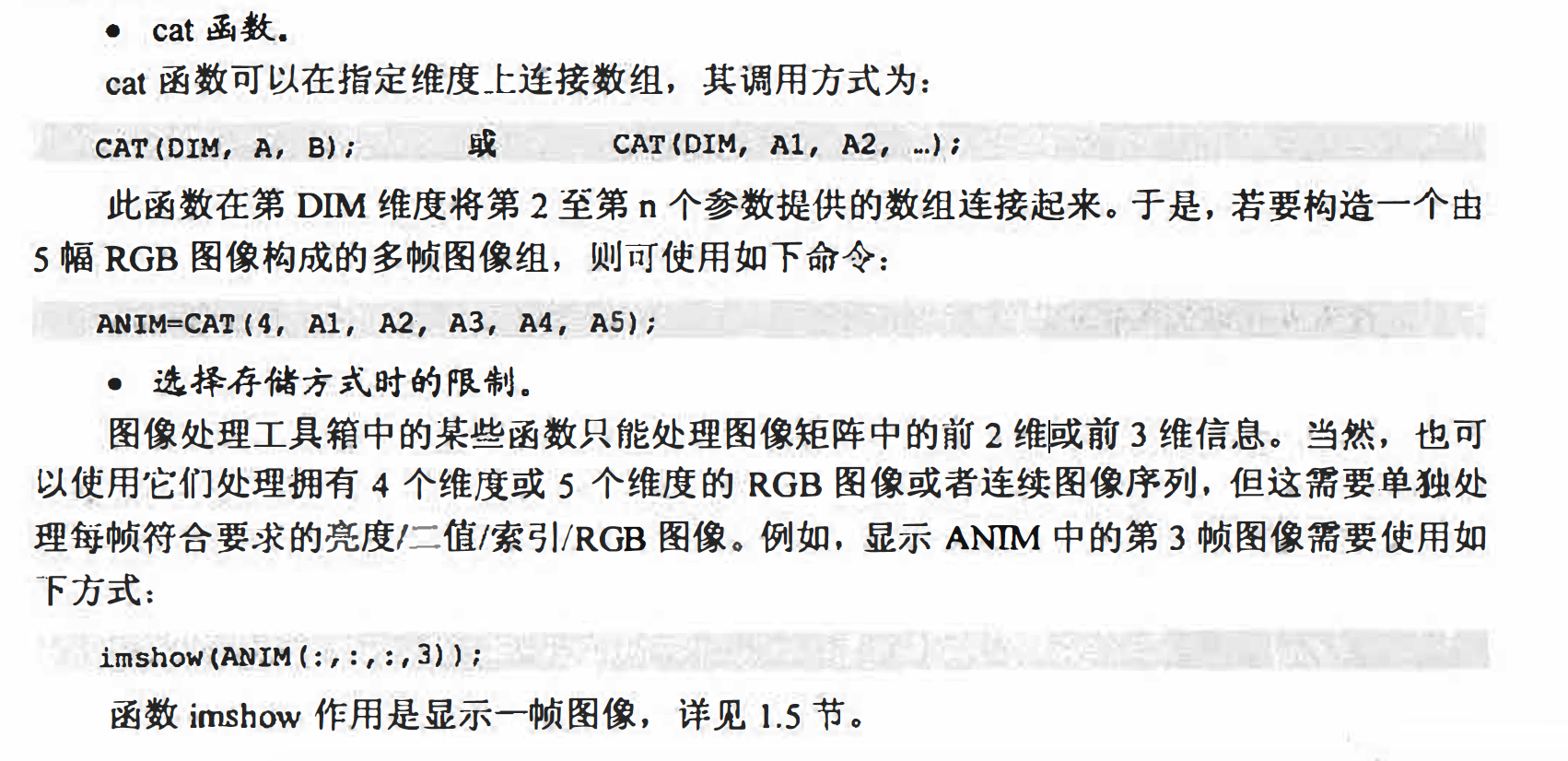默认情况下，matlab将绝大多数数据存储为双精度类型〈ω 位浮点数）以保证运算的精
确性． 而对于图像而言， 这种数据类型在图像尺寸较大时可能并不理想。例如， 一张1000
像萦见方的图像拥有一百万个像素， 如果每个像索用64位二进制数表示， 则总共需要大约
8MB的内存空间．
为了减小图像信息的空间开销， 可以将图像信息存为8 位无符号整型数（uint8）和16
位无符号整型数（ uint16 ）的数组， 这样只需要双精度浮点数八分之一或四分之一的空间。
在上述3种存储类型中双精度和 uint8 使用最多， uint16 的情况与 uint8 大致类似．

Matlab的图像转换

有时必须将图像存储格式加以转换才能使用某些图像处理函数。例如，当使用某些Matlab
内置的滤镜时， 需要将索引图像转换为RGB 图像或者灰度图像， Matlab 才会将图像滤镜应
用于图像数据本身， 而不是索引图像中的颜色索引值表〈这将产生无意义的结果〉．
Matlab 提供了一系列存储格式转换函数，如表1.11 所示．它们的名字都便于记忆，例如，
ind2gray 可以将索引图像转化为灰度图像。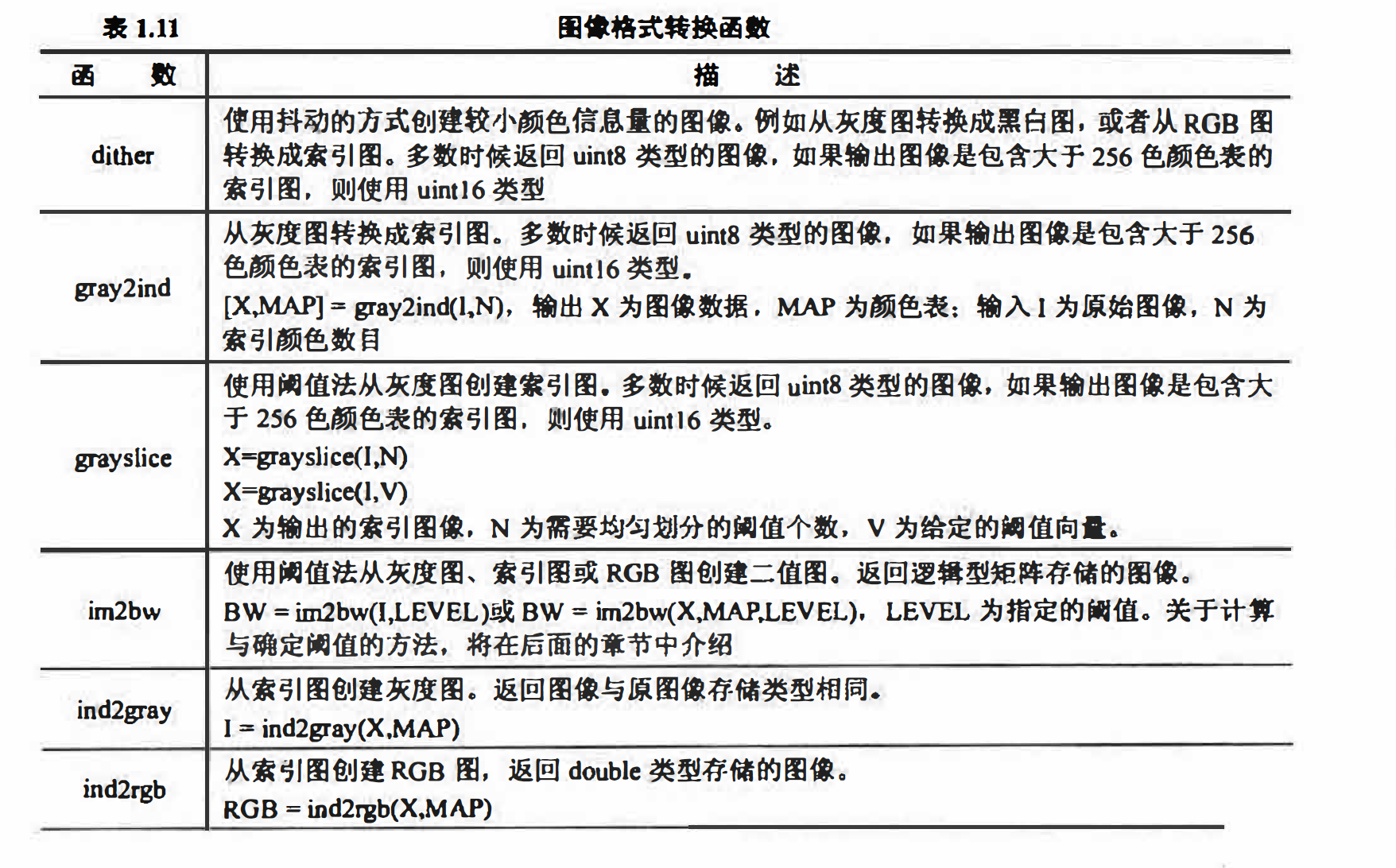也可以使用一些矩阵操作函数实现某些格式转换。例如．下面的语句可以将一幅灰度图
像转换为RGB图像．
RGBIMAGE = CAT(3, GRAY, GRAY, GRAY);

2. 图像数据类型转换
图像文件一般都为uint8类型。然而， 很多数学函数如sin等并不支持double以外的类
型， 例如， 当试图对uint8 类型直接使用sin函数进行操作时， Matlb会提示如下的错
误信息

sin(D):
H? Undehned functioii <!>i; method ’sin’ for; input a,rguments of type- ’uint8'

针对这种情况， 除了使用1.1.4 小节介绍的强制类型转换方法外， 还可利用图像处理工
具箱中的内置图像数据类型转换函数． 内置转换函数的优势在于它们可以帮助处理数据偏移
量和归一化变换， 从而简化了编程工作．
一些常用的图像类型转换函数如表1.12所示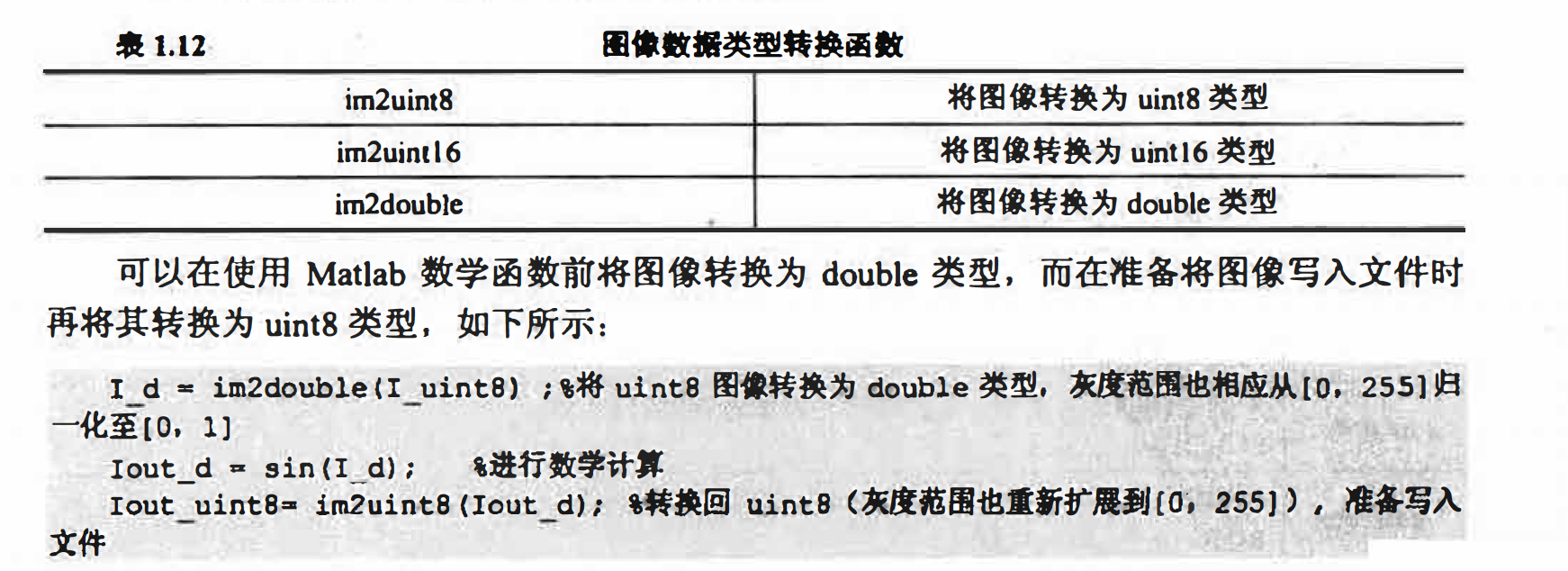图像的输入输出和显示

matlab可以处理以下的图像文件类型： BMP、HDF、JPEG、PCX、TIFF、XWD、ICO、
获得数字图像的相关信息．

型为：
A =imread(FileNAME, FMT);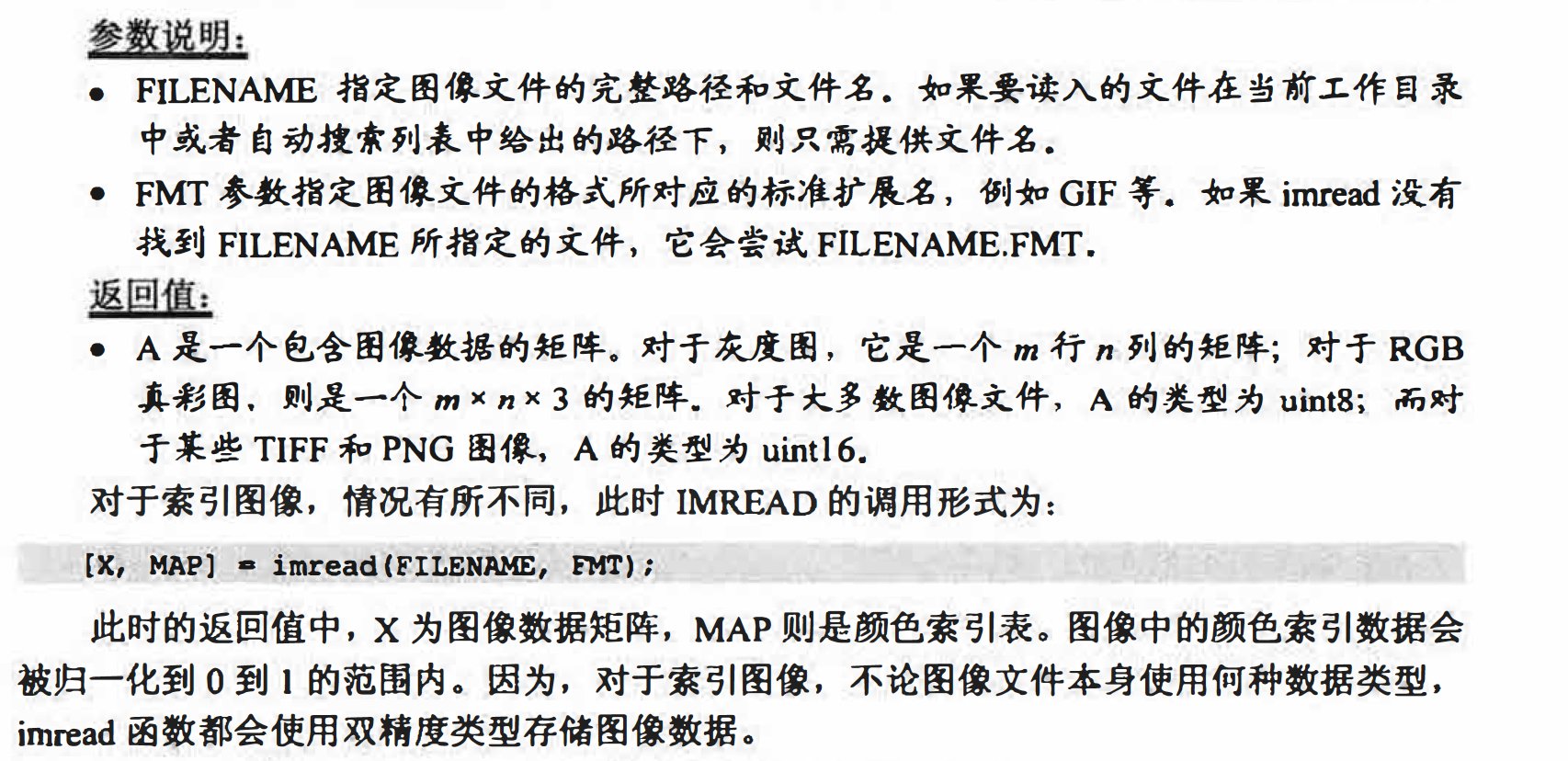2. IMWRITE函数
imwrite函数用于将指定的图像数据写入文件中，通过指定不同的保存文件扩展名，起到
图像格式转换的作用〈参见例2.4 ）. 其调用格式为：
imwite(A, FileName，FMT);
• FILENAME参数指定文件名（不必包含扩展名）．
• FMT参数指定保存文件所采用的格式．

存储索引图像时，还需要一并存储颜色索引表，则此时IMWRITE函数的使用方法应为：
imwrite(A, HAP, FILENAME, FMT);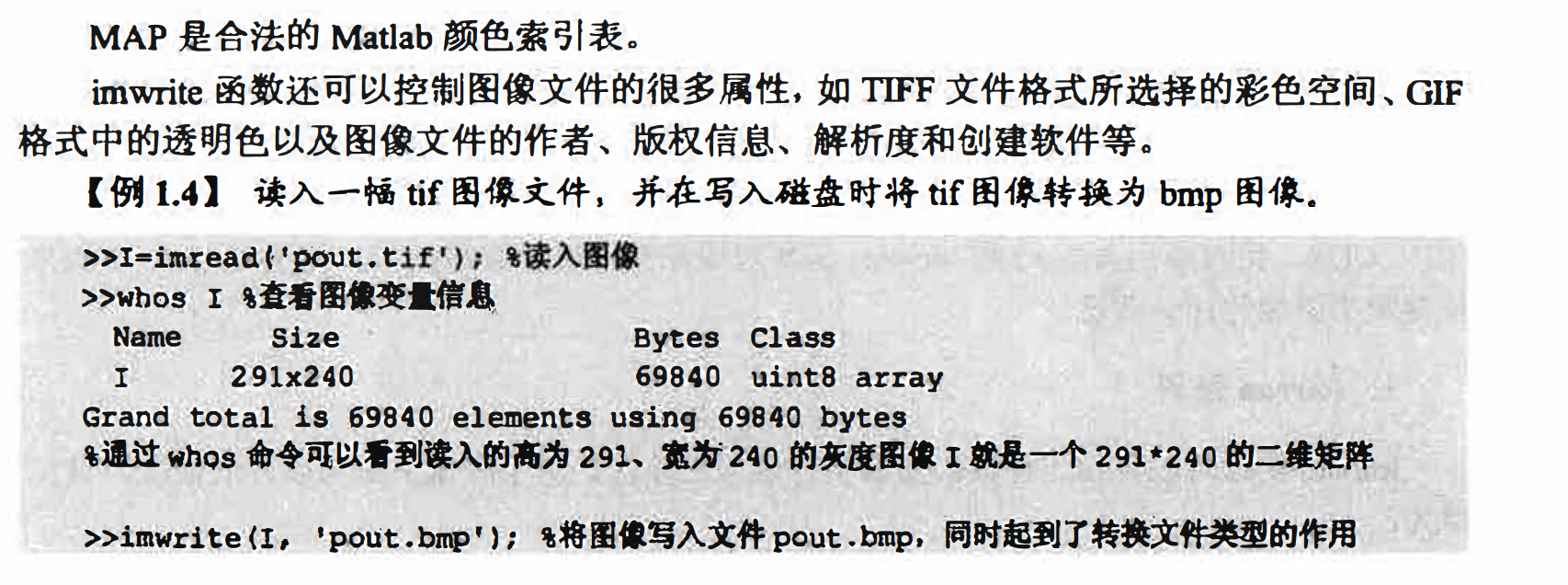3. imfinfo函数
imfinfo函数可以读取图像文件中的某些属性信息〈比如修改日期、大小、
格式、高度、宽度、色深、颜色空间、存储方式等。其调用格式为：
imfinfo(FileName,FMT);
参数说明
• FILENAME 参数指定文件名．
• FMT 参数是可选参数， 用于指定文件格式．

图像的显示
一般使用imshow函数来显示图像， 该函数可以创建一个图像对象， 并可以自动设置图
像的诸多属性，从而简化编程操作。这里介绍imshow函数的几种常见调用方式．

1.imshow函数
imshow函数用于显示工作区或图像文件中的图像．在显示的同时可控制部分效果〈参见
例12.6）， 常用的调用形式为：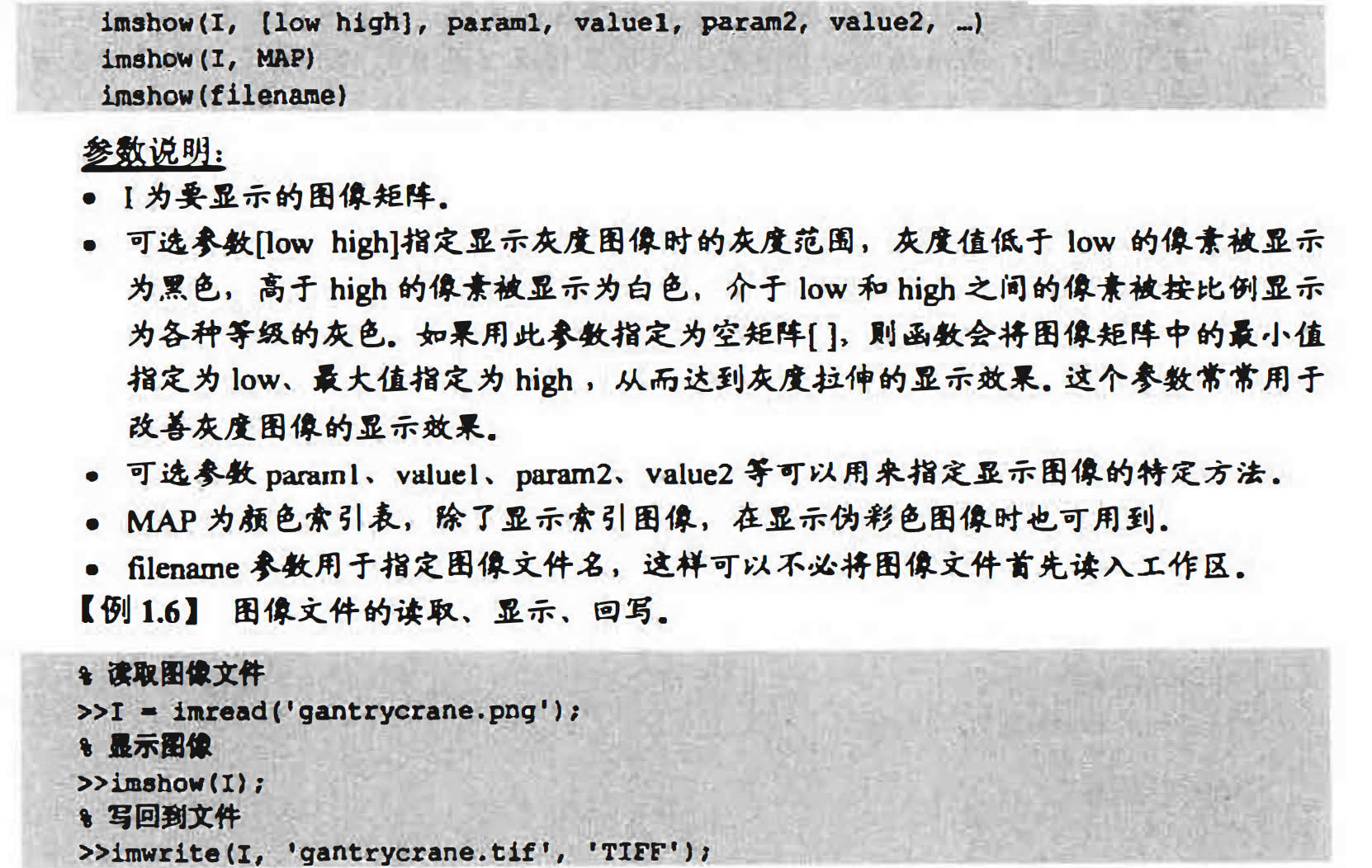2.多帧图像的显示
在显示多帧图像时，可以显示多帧中的一帧，或者将它们显示在同一个窗口内，也可以
将多帧图像转化成电影播放出来． 这3种方式的实现如例1.8所示。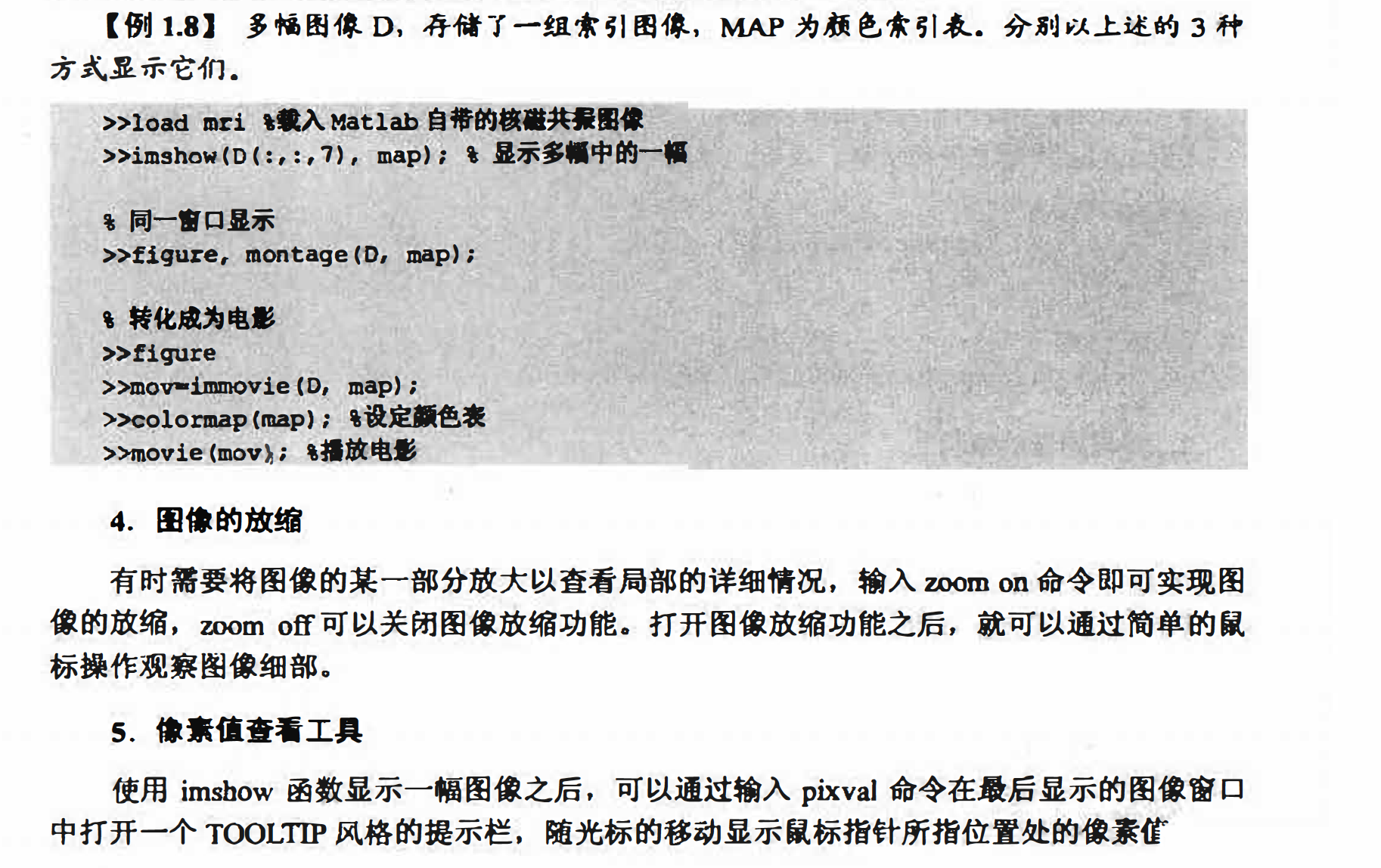展开全文图像处理 matlab 数据 class
• 标准图像显示技术特殊图象显示技术 图像运算 代数运算空间变换图像配准 图像数据变换 二维傅立叶 离散余弦 其他变换  1）radon变换： 2）fanbeam变换：   图像分析与增强 像素值及统计 1） 2）improf
matlab一些指令，便于后期查询

这些知识感觉学完还是记不住，这里自己总结了哈，希望能够加强记忆

图象显示

标准图像显示技术特殊图象显示技术

图像运算

代数运算
a.图像相减取绝对值差：K=imabsdiff(I,J)
b.图像求补：h=imcomplement(I)
空间变换
a.缩放图像
imresize
b.旋转图像
imrotate
c.修建图像
mcrop
d.空间变换
imtransform
图像配准

图像数据变换

二维傅立叶
iff2

离散余弦
a.   dct2函数
b.    dctmtx函数
其他变换
2）fanbeam变换：

图像分析与增强

A像素值及统计

1）impixel ：计算像素数据值
2）improfile：计算和绘制图像中一条或多条线段上的所有象素的灰度值（沿图像路径数据值）
3)   contour（I,N）：（中文意思为轮廓）：这个函数显示灰度图的等值线轮廓，能够自动设置坐标对象与显示图像相匹配,这里N指定了等高线的条数
4)  imhist:    （图像柱状图【直方图】），显示索引图或灰度图的灰度分布，索引的图像只支持一个图层，并且只有一个索引彩色通道。
5)  其他统计方式
B 图像分析
MATLAB图像分析技术可以提取图像的结构信息，利用edge探测边界

首先回顾一下边缘检测的一般步骤：

边缘检测算法一般包含如下四个步骤：

1.滤波（去噪）

2.增强（一般是通过计算梯度幅值）

3.检测（在图像中有许多点的梯度幅值会比较大，而这些点并不都是边缘，所以应该用某种方法来确定边缘点，比如最简单的边缘检测判据：梯度幅值阈值）

4.定位（有的应用场合要求确定边缘位置，可以在子像素水平上来估计，指出边缘的位置和方向）

边缘检测方法比较常用的有基于各种算子的方法，有基于一阶导数的各种算子（Roberts、Sobel、Prewitt等），还有基于二阶导数的拉普拉斯算子等。其中一阶导数一般找梯度极大值。二阶导数找过零点（需要忽略无意义的过零点（即均匀零区））。

1) 灰度图像的边缘检测：edge函数
BW=edge(I,method,thresh,direction/sigma)
其中method可以取‘sobel’【注：在边缘检测中，常用的一种模板是Sobel
算子。Sobel 算子有两个，一个是检测水平边缘的 ;另一个是检测垂直边缘的 】
'prewitt'【注：Prewitt算子是在图像空间利用两个方向模板与图像进行邻域卷积来完成的，这两个方向模板一个检测水平边缘，一个检测垂直边缘。】
'roberts'【注：Roberts边缘算子是一个2x2的模板，采用的是对角方向相邻的两个像素之差。从图像处理的实际效果来看，边缘定位较准，对噪声敏感。】
'log'【注：用高斯滤波器的拉普拉斯运算对I进行滤波，然后通过寻找0相交检测边缘】
‘zerocross’【注：用指定的滤波器对I滤波厚，寻找0相交边缘检测】
'canny'【 用高斯滤波器计算得到的局部最大梯度来检测边缘】
'thresh'【注：用于指定灵敏阈值】
‘sigma’【指定标准差】
小结：在上面的方法中，canny边缘检测比较清晰，log与zerocross除了有canny检测的轮廓，还有其他的杂边，而剩下边缘检测方法边检测不太全阿。
2）四叉树分解：qtdecomp函数（这部分转自uleen博客）

一个简单的示例（来自MATLAB help for qtdecomp）：
原始矩阵：
I =
1    1    1    1    2    3    6    6
1    1    2    1    4    5    6    8
1    1    1    1    7    7    7    7
1    1    1    1    6    6    5    5
20   22   20   22    1    2    3    4
20   22   22   20    5    4    7    8
20   22   20   20    9   12   40   12
20   22   20   20   13   14   15   16
>> S = qtdecomp(I,.05) %执行四叉分解，阈值为0.05,实际上的阈值是ceil(0.05*255)=13,因为I是uint8类的
S =
(1,1)        4
(5,1)        4
(1,5)        4
(5,5)        2
(7,5)        2
(5,7)        2
(7,7)        1
(8,7)        1
(7,8)        1
(8,8)        1
S是一个稀疏阵，全显示为：
>> disp(full(S));
4     0     0     0     4     0     0     0
0     0     0     0     0     0     0     0
0     0     0     0     0     0     0     0
0     0     0     0     0     0     0     0
4     0     0     0     2     0     2     0
0     0     0     0     0     0     0     0
0     0     0     0     2     0     1     1
0     0     0     0     0     0     1     1

过程：
①②③含义：3）获得四叉树分解块值：qtdgetblk函数

4)设置四叉树分解块值：qtsetblk函数
C 图像调整

图像调整技术用于图像的改善，包括提高图像信噪比、通过修正图像的颜色和灰度使某些特征更容易识别。
（通过调节灰度范围数据，来实现增大或缩小图像对比度效果）

2)使用直方图调整灰度(histeq)
(首先进行直方图均衡，再通过直方图均衡转换灰度图像的亮度值或索引值图像的颜色图值来增强图像对比度，输出图像的直方图近似于给定直方图)
3）图像色彩加强(decorrstretch)

D 图像平滑

目的：减少噪声
3种方法：
1）线性滤波
线性滤波可以从图像中去除特定成分噪音，用imfilter函数实现线性滤波

B=imfilter(A,H)         其中H为滤波器类型
fspecial：用于建立预定义的滤波算子，其语法格式为：H = fspecial(type)
H = fspecial(type，para)其中type指定算子的类型，para指定相应的参数；type可以为以下几种：（这部分粘贴来自百度）

'average'

averaging filter

为均值滤波，参数为hsize代表模板尺寸，默认值为[3，3]。

H = FSPECIAL('average',HSIZE) returns an averaging filter H of size

HSIZE. HSIZE can be a vector specifying the number of rows and columns in

H or a scalar, in which case H is a square matrix.

The default HSIZE is [3 3].

折叠'disk'

circular averaging filter

H = FSPECIAL('disk',RADIUS) returns a circular averaging filter

(pillbox) within the square matrix of side 2*RADIUS+1.

折叠'gaussian'

Gaussian lowpass filter

为高斯低通滤波，有两个参数，hsize表示模板尺寸，默认值为[3 3]，sigma为滤波器的标准值，单位为像素，默认值为0.5.

H = FSPECIAL('gaussian',HSIZE,SIGMA) returns a rotationally

symmetric Gaussian lowpass filter

of size HSIZE with standard

deviation SIGMA (positive). HSIZE can be a vector specifying the

number of rows and columns in H or a scalar, in which case H is a

square matrix.

The default HSIZE is [3 3], the default SIGMA is 0.5.

折叠'laplacian'

filter approximating the 2-D Laplacian operator

为拉普拉斯算子，参数alpha用于控制算子形状，取值范围为[0，1]，默认值为0.2.

H = FSPECIAL('laplacian',ALPHA) returns a 3-by-3 filter

approximating the shape of the two-dimensional Laplacian

operator. The parameter ALPHA controls the shape of the

Laplacian and must be in the range 0.0 to 1.0.

The default ALPHA is 0.2.

折叠'log'

Laplacian of Gaussian filter

为拉普拉斯高斯算子，有两个参数，hsize表示模板尺寸，默认值为[3 3]，sigma为滤波器的标准差，单位为像素，默认值为0.5.

H = FSPECIAL('log',HSIZE,SIGMA) returns a rotationally symmetric

Laplacian of Gaussian filter of size HSIZE with standard deviation

SIGMA (positive). HSIZE can be a vector specifying the number of rows

and columns in H or a scalar, in which case H is a square matrix.

The default HSIZE is [5 5], the default SIGMA is 0.5.

折叠'motion'

motion filter

为运动模糊算子，有两个参数，表示摄像物体逆时针方向以theta角度运动了len个像素，len的默认值为9，theta的默认值为0;

H = FSPECIAL('motion',LEN,THETA) returns a filter to approximate, once

convolved with an image, the linear motion of a camera by LEN pixels,

with an angle of THETA degrees in a counter-clockwise direction. The

filter becomes a vector for horizontal and vertical motions.

The

default LEN is 9, the default THETA is 0, which corresponds to a

horizontal motion of 9 pixels.

折叠'prewitt'

Prewitt horizontal edge-emphasizing filter

用于边缘增强，大小为[3 3]，无参数

H = FSPECIAL('prewitt') returns 3-by-3 filter that emphasizes

horizontal edges by approximating a vertical gradient. If you need to

emphasize vertical edges, transpose the filter H: H'.

[1 1 1;0 0 0;-1 -1 -1].

折叠'sobel'

Sobel horizontal edge-emphasizing filter

用于边缘提取，无参数

H = FSPECIAL('sobel') returns 3-by-3 filter that emphasizes

horizontal edges utilizing the smoothing effect by approximating a

vertical gradient. If you need to emphasize vertical edges, transpose

the filter H: H'.

[1 2 1;0 0 0;-1 -2 -1].

折叠'unsharp'

unsharp contrast enhancement filter

为对比度增强滤波器。参数alpha用于控制滤波器的形状，范围为[0，1]，默认值为0.2.

H = FSPECIAL('unsharp',ALPHA) returns a 3-by-3 unsharp contrast

enhancement filter. FSPECIAL creates the unsharp filter from the

negative of the Laplacian filter with parameter ALPHA. ALPHA controls

the shape of the Laplacian and must be in the range 0.0 to 1.0.

The default ALPHA is 0.2.

2）中值滤波（medfilt2）
特点：a.非线性处理方法；b.一定条件下克服了线性滤波模糊问题；c.适用滤除脉冲干扰以及图像扫描噪声；d.对于一些细节多，点、线、尖顶部多不宜用；

3）自适应滤波(wiener2)
针对高斯白噪声的去除效果最好

图像区域处理

1）区域设置
通过二值掩模来实现。
a.形状旋转法：（与图像内容和属性无关）可使用MATLAB中的impoint，imline,imrect,imeclipse,imploy,imfreehand分别用于可拉伸点，可拉伸线，矩形区域，椭圆区域，多边形区域和自由选择区域。
b.属性选择法（与图像属性有关）基于颜色使用roicolor函数
2）区域滤波
roifilt2
a.读入灰度图；b.设置处理区域；c.滤波操作

3）区域填充
roifill

形态学操作
1）图像膨胀
imdilate()

2）图像腐蚀
imerode()
3)形态学重建

颜色处理
显示颜色：
get(0，‘ScreenDepth’)

减少颜色
转换颜色


展开全文• 【实验名称】用于数字图像处理MATLAB基础知识 【实验目的】 1、学习并掌握MATLAB的基本语法和操作 2、学习用于数字图像处理MATLAB基础知识 【实验原理】 1、图像的读取A=imread(FILENAME,FMT) 2、...
【实验名称】用于数字图像处理的MATLAB基础知识

【实验目的】

1、学习并掌握MATLAB的基本语法和操作

2、学习用于数字图像处理的MATLAB基础知识

【实验原理】

2、图像的写入imwrite(A，FILENAME,FMT)

3、图像的显示imshow(I,[low high])

figure；%创建一个新的窗口

figure；subplot（m,n,p）；imshow（I）；

【实验内容】

1、打开MATLAB

2、读取图像（灰度I1、彩色I2），观察图像读取后的数据。

代码：

function ModifyI5()
clc;
%读取灰度图像（I1）和彩色图像（I2）
%由于彩色图像过大，不能直接打开
%将其按RGB三通道分开，便于观察
I2_R=I2(:,:,1);
I2_G=I2(:,:,2);
I2_B=I2(:,:,3);
End


结果：

灰度图像I1部分数据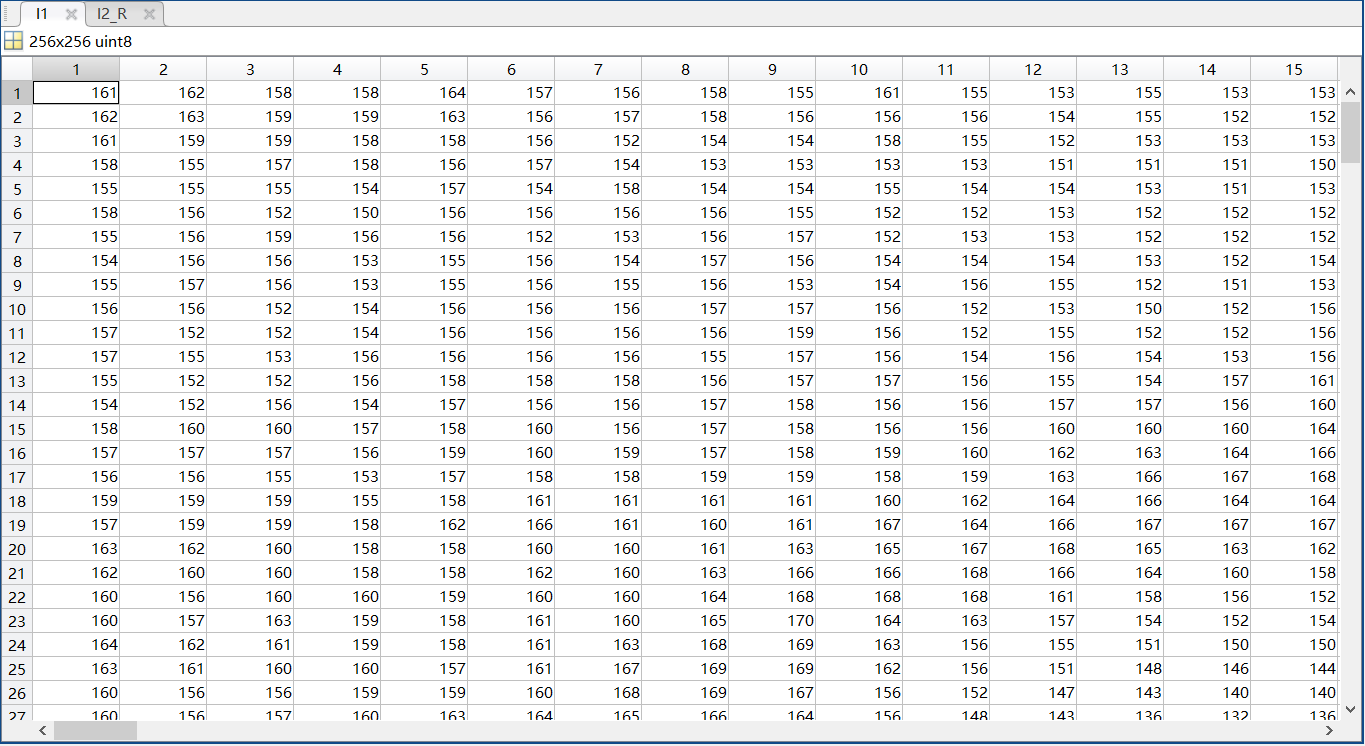彩色图像I2的R通道的部分数据：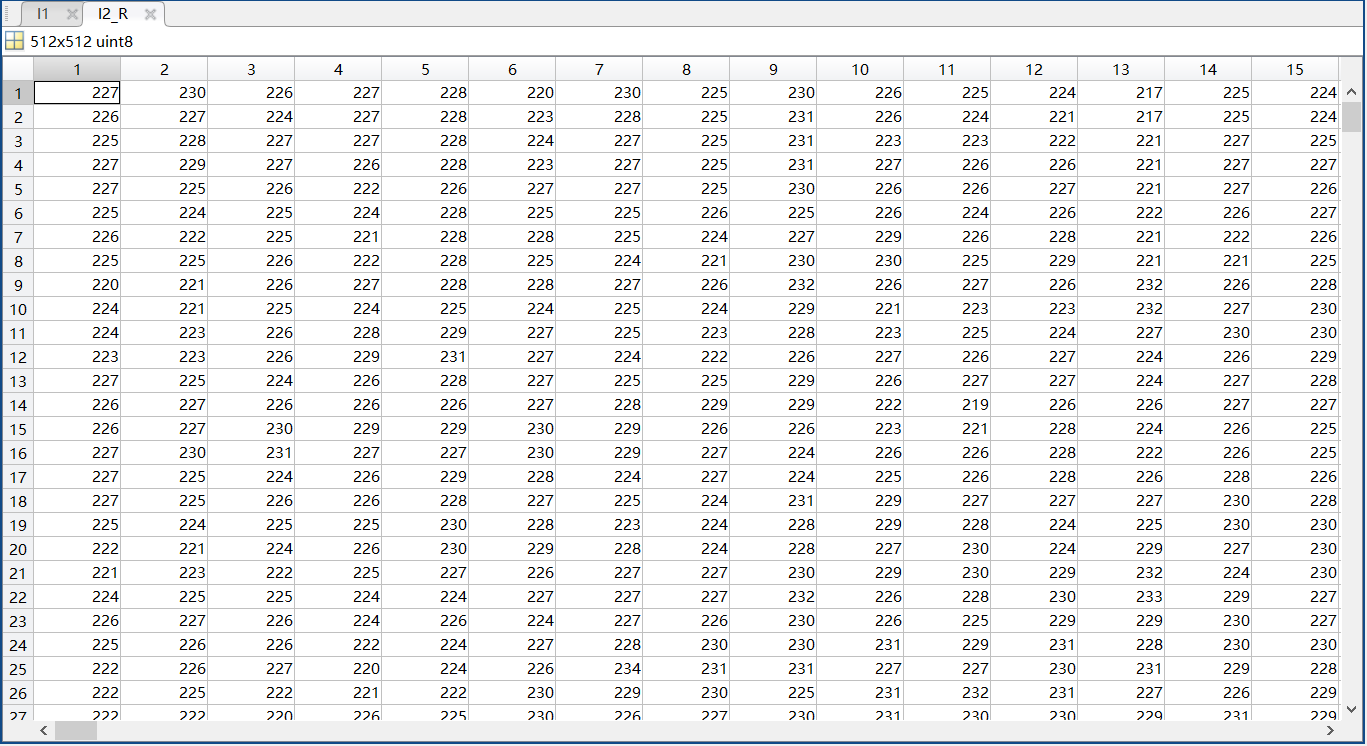3、在灰度图像中间剪切掉一块方形区域，自定义大小。

代码：

function ModifyI()
clc;
[long,width]=size(I);
%希望截取的边长，截取部分为图像正中心的以limit为边长的区域
limit=100;
%将选定区域截取并保存
i=I((long-limit)/2:(long+limit)/2,(width-limit)/2:(width+limit)/2);
%将原图截取的区域置为白色
I((long-limit)/2:(long+limit)/2,(width-limit)/2:(width+limit)/2)=255;
%绘图并保存为新图片
figure;imshow(I);imwrite(I,'new1-1.bmp');
figure;imshow(i);imwrite(i,'new1-2.bmp');
end


结果：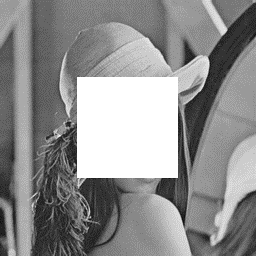截取后图像                                         截取的区域

4、在彩色图像中间剪切掉一块方形区域，自定义大小。

代码：

function ModifyI()
%希望截取的边长，截取部分为图像正中心的以limit为边长的区域
limit=100;
%将选定区域截取并保存
i(:,:,1)=I((long-limit)/2:(long+limit)/2,(width-limit)/2:(width+limit)/2,1);
i(:,:,2)=I((long-limit)/2:(long+limit)/2,(width-limit)/2:(width+limit)/2,2);
i(:,:,3)=I((long-limit)/2:(long+limit)/2,(width-limit)/2:(width+limit)/2,3);
%将原图截取的区域置为红色
I((long-limit)/2:(long+limit)/2,(width-limit)/2:(width+limit)/2,1)=255;
I((long-limit)/2:(long+limit)/2,(width-limit)/2:(width+limit)/2,2)=0;
I((long-limit)/2:(long+limit)/2,(width-limit)/2:(width+limit)/2,3)=0;
%绘图并保存为新图片
figure;imshow(I);imwrite(I,'new2-1.bmp');
figure;imshow(i);imwrite(i,'new2-2.bmp');
end


结果：截取后图像                                                                         截取的区域

5、再继续读取一幅图像I3，裁剪合适大小，填充到刚才中间剪切掉的灰度和彩色图像中。

代码：

function ModifyI()
clc;
%读取两张已经被截取过的图片和一张新灰度图片
[long1,width1]=size(I1);
[long2,width2,~]=size(I2);
[long3,width3]=size(I3);
%希望截取的边长，截取部分为图像正中心的以limit为边长的区域
limit=100;
%用新灰度图像填充灰度图像I1
I1((long1-limit)/2:(long1+limit)/2,(width1-limit)/2:(width1+limit)/2)=...
I3((long3-limit)/2:(long3+limit)/2,(width3-limit)/2:(width3+limit)/2);
%用新灰度图像填充彩色图像I2
I2((long2-limit)/2:(long2+limit)/2,(width2-limit)/2:(width2+limit)/2,1)=...
I3((long3-limit)/2:(long3+limit)/2,(width3-limit)/2:(width3+limit)/2);
I2((long2-limit)/2:(long2+limit)/2,(width2-limit)/2:(width2+limit)/2,2)=...
I3((long3-limit)/2:(long3+limit)/2,(width3-limit)/2:(width3+limit)/2);
I2((long2-limit)/2:(long2+limit)/2,(width2-limit)/2:(width2+limit)/2,3)=...
I3((long3-limit)/2:(long3+limit)/2,(width3-limit)/2:(width3+limit)/2);
%绘图并保存为新图片
figure;imshow(I1);imwrite(I1,'new1.bmp');
figure;imshow(I2);imwrite(I2,'new2.bmp');
end


结果：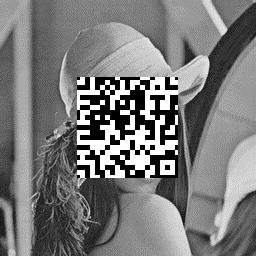填充后的灰度图片                            填充后的彩色图片

【小结或讨论】

如何彩色图像I的大小（行、列、维数）[Am,An,Az]=size(I);

如何提取彩色图像I的RGB通道像素值

I1=I(:,:,1)；I2=I(:,:,2)；I3=I（:,:,2）

如何将三个通道数据合并成一个三维矩阵

A(:,:,1)=A1；A(:,:,2)=A2；A(:,:,3)=A3

通过这次实验，我还学习了很多关于图像的基础知识。比如在观察灰度、彩色和黑白图片通道数据时，灰度图像只有一个通道，而彩色图像有RGB三个通道，如果给三个通道都赋一样的值，那么和一个通道的效果是一样的。而且要注意到灰度图像和黑白图像是不同的，黑白图像的数据只有0或255，而灰度图像的数据是在0至255之间，两者的共同点是都只有一个通道，这与彩色图像有着明显区别。。

展开全文信息安全 数字水印 信息隐藏
• 一、图像处理预备知识1、图像处理(image processing)用计算机对图像进行分析，以达到所需结果的技术。又称影像处理。图像处理一般指数字图像处理。在计算机中，按照颜色和灰度的多少可以将图像分为二值图像、灰度...
一、图像处理预备知识

1、图像处理(image processing)

用计算机对图像进行分析，以达到所需结果的技术。又称影像处理。图像处理一般指数字图像处理。

在计算机中，按照颜色和灰度的多少可以将图像分为二值图像、灰度图像、索引图像和真彩色RGB图像四种基本类型。

2、图像处理常用方法

1）图像变换

为了用正交函数或正交矩阵表示图像而对原图像所作的二维线性可逆变换。一般称原始图像为空间域图像，称变换后的图像为转换域图像，转换域图像可反变换为空间域图像。

它可以分为四个部分：空域变换等维度算法，空域变换变维度算法，值域变换等维度算法和值域变换变维度算法。其中空域变换主要指图像在几何上的变换，而值域变换主要指图像在像素值上的变换。等维度变换是在相同的维度空间中，而变维度变换是在不同的维度空间中，例如二维到三维，灰度空间到彩色空间。

常用的有三种变换方法：傅里叶变换、沃尔什-阿达玛变换、离散卡夫纳－勒维变换。这里只讲傅里叶变换。

2）图像增强和复原

图像增强不增加图片信息，只对感兴趣的特征进行强调突出，增加辨别能力。根据人的主观评定，具有一定的人为畸变。一般通过空间域处理和频率域处理两种方法。

图像复原技术的主要目的是以预先确定的目标来改善因散焦、像物相对运动、成像器材的图固有缺陷和外部干扰导致退化的图像。

图像增强和复原的目的是为了提高图像的质量，如去除噪声，提高图像的清晰度等。图像增强主要是一个主观过程，而图像复原则大部分是一个客观的过程。

3）图像压缩

图像压缩是一种减少描绘一幅图像所需数据量的技术和科学，它是数字图像处理领域中最有用和商业上最成功的技术之一。

图像压缩既可以是无损压缩，没有信息损失；也可以是有损压缩，不能通过解压恢复原图，牺牲准确度换来压缩能力的增加。

4）图像分割

图像分割是数字图像处理中的关键技术之一。分割将图像细分为构成它的子区域或物体。细分程度取决于要解决的问题。即将图像中有意义的特征部分提取出来，其有意义的特征有图像中的边缘、区域等，这是进一步进行图像识别、分析和理解的基础。

5）图像描述

图像描述是图像识别和理解的必要前提。作为最简单的二值图像可采用其几何特性描述物体的特性，一般图像的描述方法采用二维形状描述，它有边界描述和区域描述两类方法。

6）图像识别

图像经过某些预处理（增强、复原、压缩）后，进行图像分割和特征提取，从而进行判决分类。

二、MATLAB简介

Matlab是一个高级的矩阵/阵列语言，它包含控制语句、函数、数据结构、输入和输出和面向对象编程特点。用户可以在命令窗口中将输入语句与执行命令同步，也可以先编写好一个较大的复杂的应用程序（M文件）后再一起运行。

三、MATLAB图像处理代码

1、图像读取及保存

MATLAB的基本数据单位是矩阵，一幅图像可定义为一个二维函数f(x,y)，其中x和y是空间（平面）坐标，而在任何一对空间坐标(x,y)处的幅值f称为图像在该点处的强度或灰度。当x，y和灰度值f是有限的离散数值时，我们称该图像为数字图像。在MATLAB中，数字图像就是一个个矩阵。

1）图像读取

举例：

2）图像保存

函数：imwrite(OutImg, ‘图像文件’)：输出内存中图像数据到文件

举例：

imwrite(OutImg,'d:\photo\yn_ln.jpg'); %将OutImg矩阵表示的图像存储到电脑D盘photo文件夹中，并命名为yn_ln的.jpg格式的图像。

3）图像显示

函数：imshow(ImgData) ：将图像文件显示到屏幕
subplot(行数，列数，区域索引);

举例：

subplot(1,1,1);imshow(InImg); %将InImg图像文件显示到屏幕上指定位置

4）图像转换

I=rgb2gray(rgbImg)： 将彩色图像转换为灰度图像，rgbImg为彩色图像bw=im2bw(Img,level)： 将灰度图像转换为二值图像，Img为灰度图像，level为0~1的灰度级阈值；原图灰度图像                                                         二值图像

2、图像增强

1）数字图像灰度增强

J=imadjust(I,[low high], [bottom top],gamma): 对灰度图像进行直接灰度变换；I为增强前灰度图像，J为增强后的灰度图像，[low high]增强前灰度级范围，[bottom top]增强后灰度级范围

举例：

clear;
I=rgb2gray(InImg);
bw1=im2bw(I,0.8);
bw2=im2bw(J,0.8);
bw2=medfilt2(bw2);
subplot(1,2,1);imshow(bw1);
subplot(1,2,2);imshow(bw2);增强前                                                                                              增强后

2）数字图像空域滤波

S=medfilt2(J): 对图像进行中值滤波
举例：

M=0;V=0.01;
J1=imnoise(I, 'gaussian',M,V);
S=medfilt2(J1);
subplot(1, 2, 1); imshow(J1);
subplot(1, 2, 2); imshow(S);高斯噪声                                                                                        中值滤波

3）数字图像频域滤波

F=fft2(I): 2D 傅里叶正变换

Pha=angle(F): 计算相位谱

R= abs(F): 计算功率谱F1=real(F): 获得傅里叶变换实部分量F2=imag(F): 获得傅里叶变换虚部分量fftshift(F): 移动傅里叶频谱中心至零频率

I=ifft2(F): 2D 傅里叶逆变换

举例：（1）

I=BWBand(21,31);
F=fft2(I);                        %傅里叶正变换
F=fftshift(F);
L=abs(F);
L= mat2gray(L);
I3=imrotate(BWBand(21, 31),30);
F3=fft2(I3);
F3=fftshift(F3);
L3=abs(F3);
L3= mat2gray(L3);
subplot(141); imshow(I);
title('原图');
subplot(142); imshow(I3);
title('旋转30度图');
subplot(143); imshow(L);
title('功率谱图');
subplot(144); imshow(L3);

title('旋转30度功率谱图');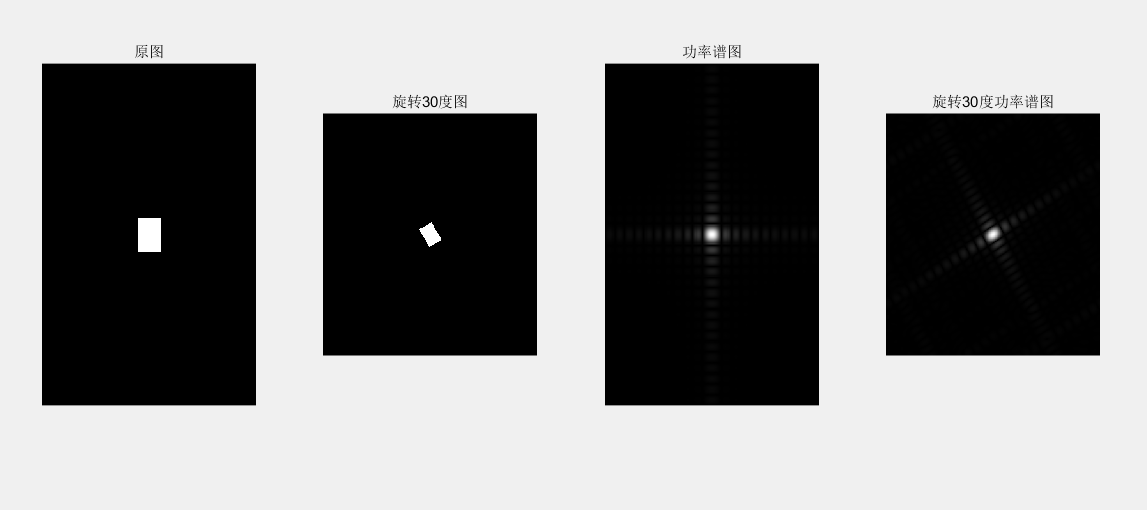subplot(2, 2, 1); imshow(InImg);
title('原彩色图');
I=rgb2gray(InImg);
J1=imnoise(I, 'salt & pepper',0.01);
subplot(2, 2, 2); imshow(J1);
title('椒盐噪声污染图');
[f1, f2]=freqspace([199 200],'meshgrid');        %产生等间隔采样的频率响应
R=sqrt(f1.^2+ f2.^2);                   %计算功率谱
Hd=ones([199 200]);
Hd(R>0.5)=0;                                   %产生理想的带通滤波器，频率带宽[0.1 0.5]
win= fspecial('gaussian',[199 200],2); %采用高斯函数生成同样大小的窗函数
win= win./max(win(:));                       %窗口函数归一化
H=fwind2(Hd,win);                           %利用窗口函数产生实际的带通滤波器
subplot(2, 2, 3); freqz2(H);
ff= imfilter (J1,H);
subplot(2, 2, 4); imshow(ff);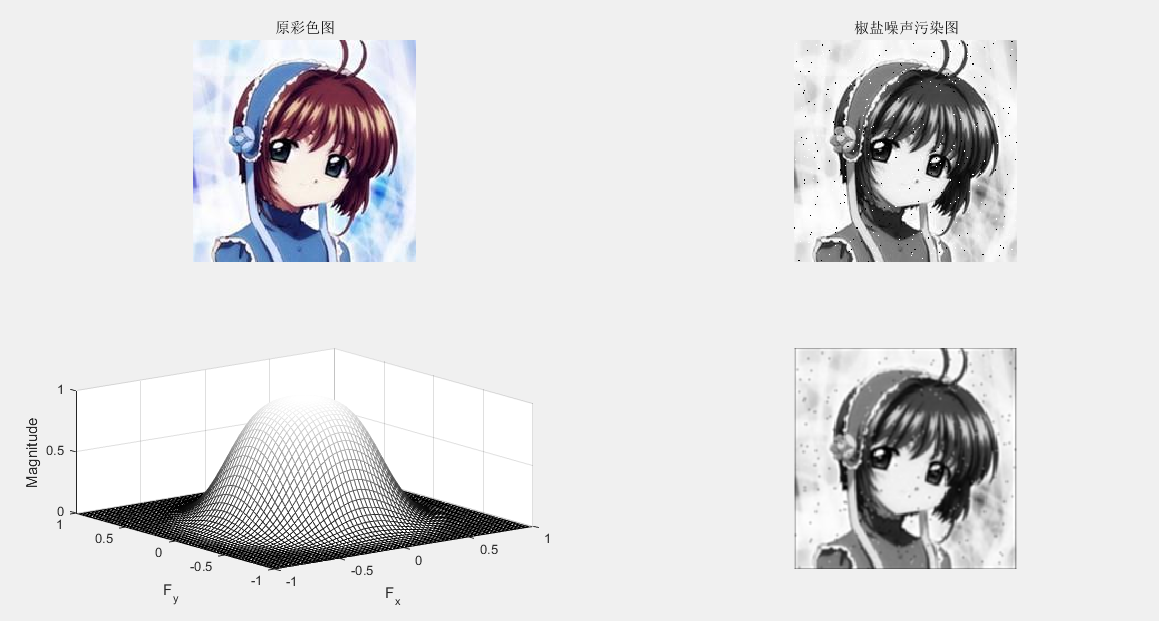3、图像复原

图像滤波：
J = WIENER2(I,[M N]): 对灰度图像实施维纳自适应滤波 ，[M N]像素领域大小C=conv2(A,B) : 计算矩阵 A 和 B 的卷积

举例：

I=rgb2gray(InImg);
J1=imnoise(I, 'gaussian');
B1=[0 0.2 0;0.2 0.2 0.2; 0 0.2 0];   %平滑滤波器
H = fspecial('average',[5 5]);
Blurred1 = imfilter (J1,H);
H = fspecial('gaussian',[5 5]);
Blurred2 = imfilter (J1,H);
K = wiener2(J1,[5 5]);
subplot(1, 5, 1); imshow(J1) ; %显示噪声图像
title('高斯噪声图像');
subplot(1, 5, 2); imshow(Blurred1) ; %显示均值滤波图像
title('均值滤波图像');
subplot(1, 5, 3); imshow(Blurred2) ; % 显示高斯滤波图像
title('高斯滤波图像');
subplot(1, 5, 4); imshow(K);% 显示维纳滤波器滤波图像
title('维纳滤波器滤波图像');
C1=conv2(J1,B1, 'same'); %对加噪后的图像平滑滤波
C1=mat2gray(C1); %将矩阵转换为灰度图像
subplot(1, 5, 5); imshow(C1);% 显示平滑图像
title('平滑图像');4、图像压缩

哈夫曼编码：无损压缩

步骤：1）缩减信源 2）尽可能少的调动信源符号排序 3）规定大概率事件编码

clear
close all;  %定义HufData/Len为全局变量的结构体
global HufData;
global Len;
disp('计算机正在准备输出霍夫曼编码结果,请耐心等待……'); %原始码字的灰度
a=rgb2gray(a);
figure;
subplot(1,2,1); imshow(a);
%取消坐标轴和边框
axis off
box off
title('Tokyo Towers','fontsize',13);
subplot(1,2,2);
axis off
box off
imhist(a);
title('图像灰度直方图','fontsize',13);%图像的灰度统计
GrayStatistics=imhist(a);
GrayStatistics=GrayStatistics';
GrayRatioo=GrayStatistics/sum(GrayStatistics);
GrayRatioNO=find(GrayRatioo~=0);
GrayRatioyes=find(GrayRatioo==0);
Len=length(GrayRatioNO);  %初始化灰度集，防止系统随即赋予其垃圾值
GrayRatio=ones(1,Len);
for i=1:Len
GrayRatio(i)=GrayRatioo(i);
end
GrayRatio=abs(sort(-GrayRatio));
for i=1:Len
HufData(i).value=GrayRatio(i); %将图像灰度概率赋予结构体
end  % 霍夫曼编码/霍夫曼编码
HuffmanCode(Len); %输出码字
lavg1=0;
for i=1:Len
tmpData=HufData(i).code
str='';
for j=1:length(tmpData) ;
str=strcat(str,num2str(tmpData(j)));
disp(strcat('a','= ',str));
end
len1=length(tmpData) ;
lavg1=lavg1+GrayRatio(1,i)*len1;

end
ratio_delet=8/lavg1;
str='';
str=strcat(str,num2str(ratio_delet));

disp(strcat('压缩率','= ',str))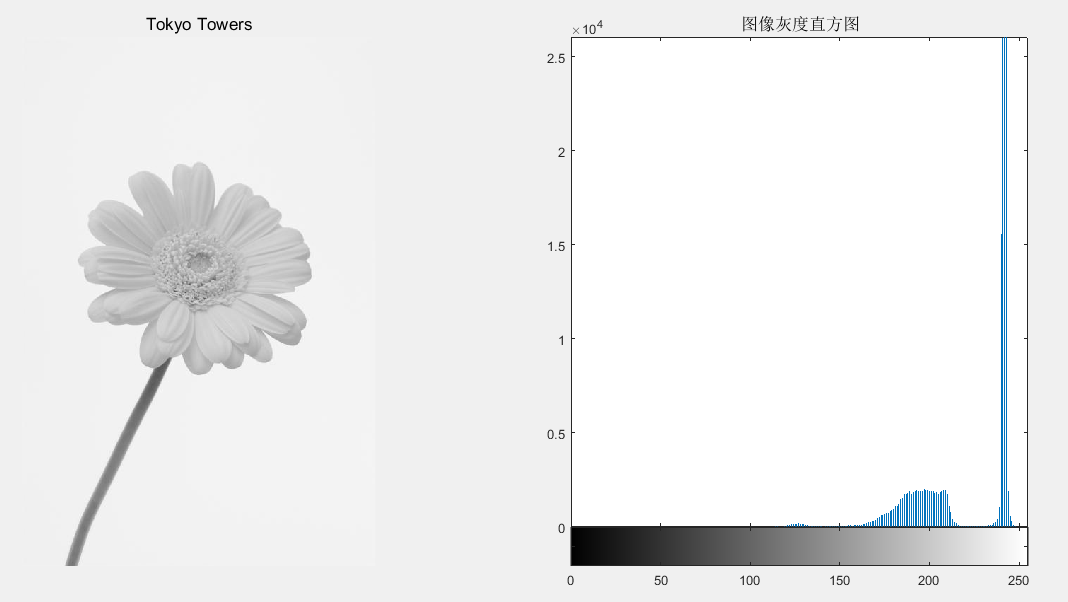5、图像分割（边缘提取）

1）图像几何纠正、边缘检测

B=imresize(A,m,type): 图像大小调整

B=imrotate(A,angle,method): 图像旋转调整

最近邻插值法：将变换后的图像中的原像素点最邻近像素的灰度值赋给原像素点的方法
双线性插值法：核心思想是在两个方向分别进行一次线性插值。

举例：

I=rgb2gray(InImg);
B1=imresize(I,1.5, 'nearest');
B2=imresize(I,1.5, 'bilinear');
C1= imrotate (I,45, 'nearest');
C2= imrotate (I,45, 'bilinear');
subplot(231);imshow(I) ;%显示原始图像于
title('原始图像');
subplot(232); imshow(B1) ;%显示调整图像(最近邻插值)
title('最近邻插值图像');
subplot(233); imshow(B2) ;% 显示调整图像(双线性插值)
title('双线性插值图像');
subplot(234); imshow(C1);% 显示旋转图像(最近邻插值)
title('旋转最近邻插值图像');
subplot(235); imshow(C2);% 显示旋转图像(双线性插值)
title('旋转双线性插值');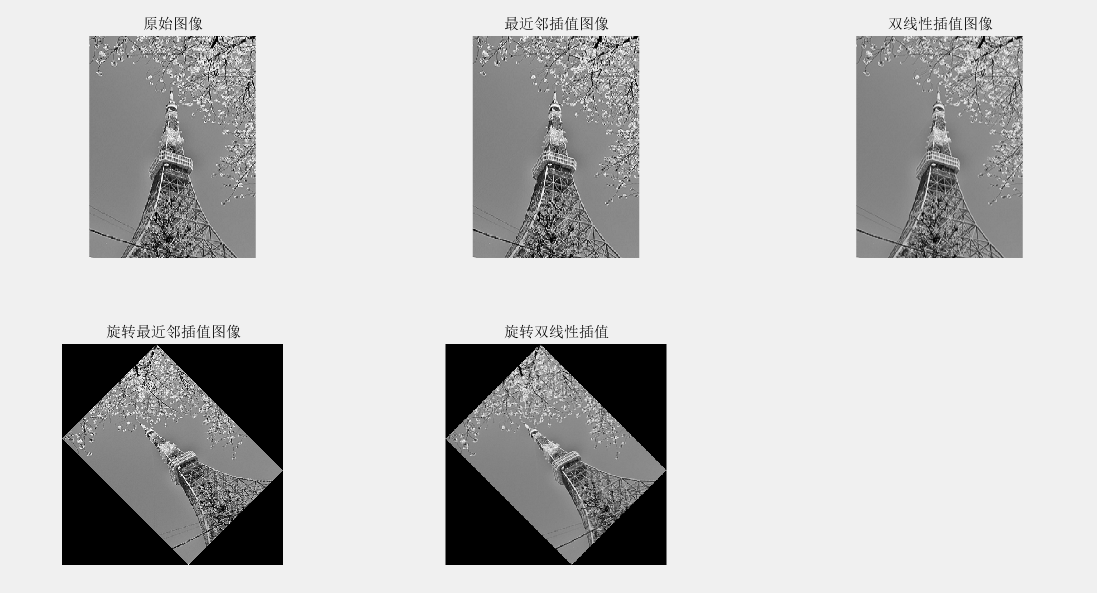2）边缘提取

BW=edge(I,method,thresh): 检测灰度图像的边缘

举例：

I=rgb2gray(InImg);
BW1= edge (I, 'sobel',0.1);%sobel边缘检测器
BW2= edge (I, 'prewitt',0.1);%prewitt边缘检测器
BW3= edge (I, 'roberts',0.1);%roberts边缘检测器
BW4= edge (I, 'log',0.01,2.0);%log边缘检测器
subplot(221); imshow(BW1) ;% 显示边缘图像
title('sobel边缘图像');
subplot(222); imshow(BW2) ;%显示边缘图像
title('prewitt边缘图像');
subplot(223); imshow(BW3) ;% 显示边缘图像
title('roberts边缘图像');
subplot(224); imshow(BW4) ;% 显示边缘图像
title('log边缘图像');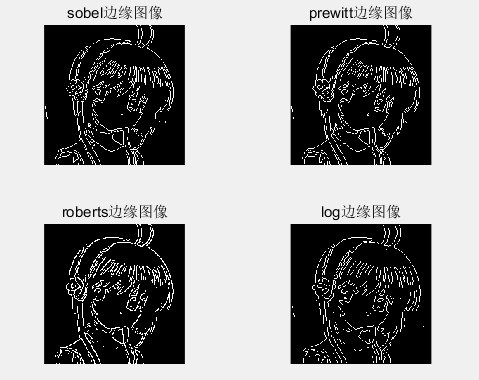边缘提取


展开全文• 显示索引图像和灰度图像&gt;&gt; [X,map]=imread('trees.tif');&gt;&gt; gmap=rgb2gray(map);&gt;&gt; figure,imshow(X,map);&gt;&gt; figure,imshow(X,gmap);利用膨胀函数平移图像...
显示索引图像和灰度图像>> [X,map]=imread('trees.tif');>> gmap=rgb2gray(map);>> figure,imshow(X,map);>> figure,imshow(X,gmap);利用膨胀函数平移图像I = imread('football.jpg');se = translate(strel(1), [30 30]);%将一个平面结构化元素分别向下和向右移动30个位置J = imdilate(I,se);%利用膨胀函数平移图像subplot(121);imshow(I), title('原图')subplot(122), imshow(J), title('移动后的图像');水平翻转和上下翻转I = imread('cameraman.tif');Flip1=fliplr(I);               %  对矩阵I左右反转subplot(131);imshow(I);title('原图');subplot(132);imshow(Flip1);title('水平镜像');Flip2=flipud(I);               %  对矩阵I垂直反转subplot(133);imshow(Flip2);title('竖直镜像');图像旋转I=imread('cameraman.tif');B=imrotate(I,60,'bilinear','crop');%双线性插值法旋转图像，并裁剪图像，使其和原图像大小一致subplot(121),imshow(I),title('原图');subplot(122),imshow(B),title('旋转图像60^{o}，并剪切图像');截取图像I = imread('circuit.tif');I2 = imcrop(I,[75 68 130 112]);imshow(I), figure, imshow(I2)画轮廓>> I=imread('circuit.tif');>> imshow(I)>> figure>> imcontour(I,3)噪声和滤波I=imread('cameraman.tif');J=imnoise(I,'salt & pepper',0.02);%添加椒盐噪声subplot(121),imshow(J);title('噪声图像')K=medfilt2(J);%使用3*3的邻域窗的中值滤波subplot(122),imshow(K);title('中值滤波后图像')Matlab数字数字图像处理函数汇总：1、数字数字图像的变换① fft2：fft2函数用于数字数字图像的二维傅立叶变换，如：i=imread('104_8.tif');j=fft2(i);②ifft2:：ifft2函数用于数字数字图像的二维傅立叶反变换，如： i=imread('104_8.tif'); j=fft2(i);k=ifft2(j);2、模拟噪声生成函数和预定义滤波器① imnoise：用于对数字数字图像生成模拟噪声，如： i=imread('104_8.tif'); j=imnoise(i,'gaussian',0,0.02);%模拟高斯噪声② fspecial：用于产生预定义滤波器，如：h=fspecial('sobel');%sobel水平边缘增强滤波器h=fspecial('gaussian');%高斯低通滤波器h=fspecial('laplacian');%拉普拉斯滤波器h=fspecial('log');%高斯拉普拉斯（LoG）滤波器h=fspecial('average');%均值滤波器2、数字数字图像的增强①直方图：imhist函数用于数字数字图像的直方图显示，如：i=imread('104_8.tif');imhist(i);②直方图均化：histeq函数用于数字数字图像的直方图均化，如：i=imread('104_8.tif');j=histeq(i);imshow(J)③对比度调整：imadjust函数用于数字数字图像的对比度调整，如：i=imread('104_8.tif');j=imadjust(i,[0.3,0.7],[]);④对数变换：log函数用于数字数字图像的对数变换，如：i=imread('104_8.tif');j=double(i);k=log(j);⑤基于卷积的数字数字图像滤波函数：filter2函数用于数字数字图像滤波，如：i=imread('104_8.tif');h=[1,2,1;0,0,0;-1,-2,-1];j=filter2(h,i);⑥线性滤波：利用二维卷积conv2滤波, 如:i=imread('104_8.tif');h=[1,1,1;1,1,1;1,1,1];h=h/9;j=conv2(i,h);⑦中值滤波：medfilt2函数用于数字数字图像的中值滤波，如：i=imread('104_8.tif');j=medfilt2(i);⑧锐化（1）利用Sobel算子锐化数字数字图像, 如:i=imread('104_8.tif');h=[1,2,1;0,0,0;-1,-2,-1];%Sobel算子j=filter2(h,i);（2）利用拉氏算子锐化数字数字图像, 如:i=imread('104_8.tif');j=double(i);h=[0,1,0;1,-4,0;0,1,0];%拉氏算子k=conv2(j,h,'same');m=j-k;3、数字数字图像边缘检测①sobel算子 如：i=imread('104_8.tif');j =edge(i,'sobel',thresh) ②prewitt算子 如：i=imread('104_8.tif');j =edge(i,'prewitt',thresh)③roberts算子  如：i=imread('104_8.tif');j =edge(i,'roberts',thresh)④log算子  如：i=imread('104_8.tif');j =edge(i,'log',thresh)⑤canny算子 如：i=imread('104_8.tif');j =edge(i,'canny',thresh)⑥Zero-Cross算子 如：i=imread('104_8.tif');j =edge(i,'zerocross',thresh)4、形态学数字数字图像处理①膨胀：是在二值化数字数字图像中“加长”或“变粗”的操作，函数imdilate执行膨胀运算，如：a=imread('104_7.tif');%输入二值数字数字图像b=[0 1 0;1 1 1;01 0];c=imdilate(a,b);②腐蚀：函数imerode执行腐蚀，如：a=imread('104_7.tif');%输入二值数字数字图像b=strel('disk',1);c=imerode(a,b);③开运算：先腐蚀后膨胀称为开运算，用imopen来实现，如： a=imread('104_8.tif');b=strel('square',2);c=imopen(a,b);④闭运算：先膨胀后腐蚀称为闭运算，用imclose来实现，如： a=imread('104_8.tif');b=strel('square',2);c=imclose(a,b);  数字数字图像增强1. 直方图均衡化的 Matlab 实现1.1 imhist 函数功能：计算和显示数字数字图像的色彩直方图格式：imhist(I,n)        imhist(X,map)说明：imhist(I,n) 其中，n 为指定的灰度级数目，缺省值为256；imhist(X,map) 就算和显示索引色数字数字图像 X 的直方图，map为调色板。用stem(x,counts) 同样可以显示直方图。1.2 imcontour 函数功能：显示数字数字图像的等灰度值图格式：imcontour(I,n),imcontour(I,v)说明：n 为灰度级的个数，v 是有用户指定所选的等灰度级向量。1.3 imadjust 函数功能：通过直方图变换调整对比度格式：J=imadjust(I,[low high],[bottomtop],gamma)        newmap=imadjust(map,[low high],[bottomtop],gamma)说明：J=imadjust(I,[low high],[bottomtop],gamma) 其中，gamma 为校正量r，[lowhigh] 为原数字数字图像中要变换的灰度范围，[bottom top]指定了变换后的灰度范围；newmap=imadjust(map,[lowhigh],[bottom top],gamma) 调整索引色数字数字图像的调色板 map 。此时若 [low high] 和[bottom top] 都为2×3的矩阵，则分别调整 R、G、B 3个分量。1.4 histeq 函数功能：直方图均衡化格式：J=histeq(I,hgram)        J=histeq(I,n)        [J,T]=histeq(I,...)        newmap=histeq(X,map,hgram)        newmap=histeq(X,map)        [new,T]=histeq(X,...)说明：J=histeq(I,hgram) 实现了所谓“直方图规定化”，即将原是图象 I 的直方图变换成用户指定的向量 hgram 。hgram 中的每一个元素都在 [0,1] 中；J=histeq(I,n) 指定均衡化后的灰度级数 n ，缺省值为 64；[J,T]=histeq(I,...)返回从能将数字数字图像 I 的灰度直方图变换成数字数字图像 J 的直方图的变换 T ；newmap=histeq(X,map) 和 [new,T]=histeq(X,...) 是针对索引色数字数字图像调色板的直方图均衡。2. 噪声及其噪声的 Matlab 实现        imnoise 函数格式：J=imnoise(I,type)        J=imnoise(I,type,parameter)说明：J=imnoise(I,type) 返回对数字数字图像 I 添加典型噪声后的有噪数字数字图像 J ，参数type 和 parameter 用于确定噪声的类型和相应的参数。3. 数字数字图像滤波的 Matlab 实现3.1 conv2 函数功能：计算二维卷积格式：C=conv2(A,B)        C=conv2(Hcol,Hrow,A)        C=conv2(...,'shape')说明：对于 C=conv2(A,B) ，conv2 的算矩阵A 和 B 的卷积，若[Ma,Na]＝size(A), [Mb,Nb]=size(B), 则 size(C)=[Ma+Mb-1,Na+Nb-1];C=conv2(Hcol,Hrow,A) 中，矩阵 A 分别与Hcol 向量在列方向和 Hrow 向量在行方向上进行卷积；C=conv2(...,'shape') 用来指定 conv2返回二维卷积结果部分，参数 shape 可取值如下：        》full为缺省值，返回二维卷积的全部结果；        》same返回二维卷积结果中与 A 大小相同的中间部分；        valid 返回在卷积过程中，未使用边缘补 0 部分进行计算的卷积结果部分，当 size(A)>size(B) 时，size(C)=[Ma-Mb+1,Na-Nb+1]。3.2 conv 函数功能：计算多维卷积格式：与 conv2 函数相同3.3 filter2函数功能：计算二维线型数字滤波，它与函数 fspecial 连用格式：Y=filter2(B,X)        Y=filter2(B,X,'shape')说明：对于 Y=filter2(B,X) ，filter2 使用矩阵B 中的二维 FIR 滤波器对数据 X 进行滤波，结果 Y 是通过二维互相关计算出来的，其大小与 X 一样；对于Y=filter2(B,X,'shape') ，filter2返回的 Y 是通过二维互相关计算出来的，其大小由参数 shape 确定，其取值如下：        》full返回二维相关的全部结果，size(Y)>size(X)；        》same返回二维互相关结果的中间部分，Y 与X 大小相同；        》valid返回在二维互相关过程中，未使用边缘补 0 部分进行计算的结果部分，有 size(Y)<size(X) 。3.4 fspecial 函数功能：产生预定义滤波器格式：H=fspecial(type)        H=fspecial('gaussian',n,sigma)        高斯低通滤波器        H=fspecial('sobel')                        Sobel 水平边缘增强滤波器        H=fspecial('prewitt')                     Prewitt 水平边缘增强滤波器        H=fspecial('laplacian',alpha)            近似二维拉普拉斯运算滤波器        H=fspecial('log',n,sigma)                高斯拉普拉斯（LoG）运算滤波器        H=fspecial('average',n)                  均值滤波器        H=fspecial('unsharp',alpha)            模糊对比增强滤波器说明：对于形式 H=fspecial(type) ，fspecial 函数产生一个由 type 指定的二维滤波器 H ，返回的H 常与其它滤波器搭配使用。4. 彩色增强的 Matlab 实现4.1 imfilter函数功能：真彩色增强格式：B=imfilter(A,h)说明：将原始数字数字图像 A 按指定的滤波器 h 进行滤波增强处理，增强后的数字数字图像 B 与A 的尺寸和类型相同 数字数字图像的变换1. 离散傅立叶变换的Matlab 实现      Matlab 函数 fft、fft2 和 fftn 分别可以实现一维、二维和 N 维 DFT 算法；而函数 ifft、ifft2 和 ifftn 则用来计算反 DFT 。这些函数的调用格式如下：         A＝fft(X,N,DIM)      其中，X 表示输入数字数字图像；N 表示采样间隔点，如果 X 小于该数值，那么 Matlab 将会对 X 进行零填充，否则将进行截取，使之长度为N ；DIM 表示要进行离散傅立叶变换。        A＝fft2(X,MROWS,NCOLS) 其中，MROWS 和 NCOLS 指定对 X 进行零填充后的 X 大小。        A＝fftn(X,SIZE)其中，SIZE 是一个向量，它们每一个元素都将指定 X 相应维进行零填充后的长度。      函数 ifft、ifft2 和 ifftn的调用格式于对应的离散傅立叶变换函数一致。例子：数字数字图像的二维傅立叶频谱% 读入原始数字数字图像I＝imread('lena.bmp');imshow(I)% 求离散傅立叶频谱J=fftshift(fft2(I));figure;imshow(log(abs(J)),[8,10])2. 离散余弦变换的 Matlab实现2.1. dCT2 函数功能：二维 DCT 变换格式：B=dct2(A)         B=dct2(A,m,n)         B=dct2(A,[m,n]) 说明：B＝dct2(A) 计算 A 的 DCT 变换 B ，A 与 B 的大小相同；B＝dct2(A,m,n) 和 B=dct2(A,[m,n]) 通过对 A 补 0 或剪裁，使 B 的大小为 m×n。2.2. dict2 函数功能：DCT 反变换格式：B=idct2(A)         B=idct2(A,m,n)         B=idct2(A,[m,n]) 说明：B＝idct2(A) 计算 A 的 DCT 反变换 B ，A 与 B 的大小相同；B＝idct2(A,m,n) 和 B=idct2(A,[m,n]) 通过对 A 补 0 或剪裁，使 B的大小为 m×n。2.3. dctmtx函数功能：计算 DCT 变换矩阵格式：D＝dctmtx(n)说明：D＝dctmtx(n) 返回一个 n×n 的 DCT 变换矩阵，输出矩阵 D 为 double 类型。3. 数字数字图像小波变换的Matlab 实现3.1 一维小波变换的 Matlab实现(1) dwt 函数功能：一维离散小波变换格式：[cA,cD]=dwt(X,'wname')        [cA,cD]=dwt(X,Lo_D,Hi_D)说明：[cA,cD]=dwt(X,'wname')使用指定的小波基函数'wname' 对信号 X 进行分解，cA、cD分别为近似分量和细节分量；[cA,cD]=dwt(X,Lo_D,Hi_D) 使用指定的滤波器组 Lo_D、Hi_D 对信号进行分解。(2) idwt 函数功能：一维离散小波反变换格式：X=idwt(cA,cD,'wname')        X=idwt(cA,cD,Lo_R,Hi_R)        X=idwt(cA,cD,'wname',L)        X=idwt(cA,cD,Lo_R,Hi_R,L)说明：X=idwt(cA,cD,'wname')由近似分量 cA 和细节分量 cD 经小波反变换重构原始信号 X 。        'wname' 为所选的小波函数        X=idwt(cA,cD,Lo_R,Hi_R) 用指定的重构滤波器 Lo_R 和 Hi_R 经小波反变换重构原始信号 X 。        X=idwt(cA,cD,'wname',L) 和 X=idwt(cA,cD,Lo_R,Hi_R,L) 指定返回信号 X 中心附近的 L 个点。3.2 二维小波变换的 Matlab实现          二维小波变换的函数-------------------------------------------------     函数名                函数功能---------------------------------------------------     dwt2           二维离散小波变换   wavedec2       二维信号的多层小波分解     idwt2          二维离散小波反变换   waverec2        二维信号的多层小波重构   wrcoef2          由多层小波分解重构某一层的分解信号   upcoef2          由多层小波分解重构近似分量或细节分量   detcoef2         提取二维信号小波分解的细节分量   appcoef2        提取二维信号小波分解的近似分量   upwlev2         二维小波分解的单层重构   dwtpet2         二维周期小波变换   idwtper2        二维周期小波反变换-------------------------------------------------------------(1) wcodemat 函数功能：对数据矩阵进行伪彩色编码格式：Y=wcodemat(X,NB,OPT,ABSOL)        Y=wcodemat(X,NB,OPT)        Y=wcodemat(X,NB)        Y=wcodemat(X)说明：Y=wcodemat(X,NB,OPT,ABSOL)返回数据矩阵 X 的编码矩阵 Y ；NB 伪编码的最大值，即编码范围为 0～NB，缺省值 NB＝16；       OPT 指定了编码的方式（缺省值为 'mat'），即：                OPT＝'row' ，按行编码                OPT＝'col' ，按列编码                OPT＝'mat' ，按整个矩阵编码       ABSOL 是函数的控制参数（缺省值为 '1'），即：                ABSOL＝0 时，返回编码矩阵                ABSOL＝1 时，返回数据矩阵的绝对值ABS(X)(2) dwt2 函数功能：二维离散小波变换格式：[cA,cH,cV,cD]=dwt2(X,'wname')        [cA,cH,cV,cD]=dwt2(X,Lo_D,Hi_D)说明：[cA,cH,cV,cD]=dwt2(X,'wname')使用指定的小波基函数 'wname' 对二维信号 X 进行二维离散小波变幻；cA，cH,cV,cD 分别为近似分量、水平细节分量、垂直细节分量和对角细节分量；[cA,cH,cV,cD]=dwt2(X,Lo_D,Hi_D) 使用指定的分解低通和高通滤波器 Lo_D 和 Hi_D 分解信号 X 。(3) wavedec2 函数功能：二维信号的多层小波分解格式：[C,S]=wavedec2(X,N,'wname')        [C,S]=wavedec2(X,N,Lo_D,Hi_D)说明：[C,S]=wavedec2(X,N,'wname')使用小波基函数'wname' 对二维信号 X 进行 N 层分解；[C,S]=wavedec2(X,N,Lo_D,Hi_D) 使用指定的分解低通和高通滤波器Lo_D 和 Hi_D 分解信号 X 。(4) idwt2 函数功能：二维离散小波反变换格式：X=idwt2(cA,cH,cV,cD,'wname')        X=idwt2(cA,cH,cV,cD,Lo_R,Hi_R)        X=idwt2(cA,cH,cV,cD,'wname',S)        X=idwt2(cA,cH,cV,cD,Lo_R,Hi_R,S)说明：X=idwt2(cA,cH,cV,cD,'wname')由信号小波分解的近似信号cA 和细节信号 cH、cH、cV、cD 经小波反变换重构原信号 X；X=idwt2(cA,cH,cV,cD,Lo_R,Hi_R)使用指定的重构低通和高通滤波器 Lo_R 和 Hi_R 重构原信号 X ；X=idwt2(cA,cH,cV,cD,'wname',S)和X=idwt2(cA,cH,cV,cD,Lo_R,Hi_R,S) 返回中心附近的 S 个数据点。(5) waverec2 函数说明：二维信号的多层小波重构格式：X=waverec2(C,S,'wname')        X=waverec2(C,S,Lo_R,Hi_R)说明：X=waverec2(C,S,'wname')由多层二维小波分解的结果 C、S 重构原始信号 X ，'wname'为使用的小波基函数；X=waverec2(C,S,Lo_R,Hi_R) 使用重构低通和高通滤波器 Lo_R 和 Hi_R 重构原信号。  数字数字图像处理工具箱1. 数字数字图像和数字数字图像数据   缺省情况下，MATLAB将数字数字图像中的数据存储为双精度类型(double)，64位浮点数，所需存储量很大；MATLAB还支持另一种类型无符号整型(uint8)，即数字数字图像矩阵中每个数据占用1个字节。   在使用MATLAB工具箱时，一定要注意函数所要求的参数类型。另外，uint8与double两种类型数据的值域不同，编程需注意值域转换。          从uint8到double的转换   ---------------------------------------------       数字数字图像类型        MATLAB语句   ---------------------------------------------     索引色             B=double(A)+1     索引色或真彩色 B=double(A)/255     二值数字数字图像          B=double(A)   ---------------------------------------------         从double到uint8的转换   ---------------------------------------------       数字数字图像类型        MATLAB语句   ---------------------------------------------    索引色              B=uint8(round(A-1))    索引色或真彩色   B=uint8(round(A*255))    二值数字数字图像           B=logical(uint8(round(A)))   ---------------------------------------------2. 数字数字图像处理工具箱所支持的数字数字图像类型2.1 真彩色数字数字图像    R、G、B三个分量表示一个像素的颜色。如果要读取数字数字图像中(100,50)处的像素值，可查看三元数据(100,50,1:3)。    真彩色数字数字图像可用双精度存储，亮度值范围是[0,1]；比较符合习惯的存储方法是用无符号整型存储，亮度值范围[0,255]   2.2 索引色数字数字图像   包含两个结构，一个是调色板，另一个是数字数字图像数据矩阵。调色板是一个有3列和若干行的色彩映象矩阵，矩阵每行代表一种颜色，3列分别代表红、绿、蓝色强度的双精度数。      注意：MATLAB中调色板色彩强度[0,1]，0代表最暗，1代表最亮。          常用颜色的RGB值   --------------------------------------------    颜色   R   G   B      颜色    R  G   B    --------------------------------------------     黑     0   0   1     洋红    1   0   1     白     1   1   1     青蓝    0   1   1     红     1   0   0     天蓝 0.67 0   1     绿     0   1   0     橘黄    1 0.5 0     蓝     0   0   1     深红   0.5 0   0     黄     1   1   0      灰    0.5 0.5 0.5          --------------------------------------------         产生标准调色板的函数   -------------------------------------------------    函数名      调色板   -------------------------------------------------     Hsv       色彩饱和度，以红色开始，并以红色结束     Hot       黑色－红色－黄色－白色     Cool      青蓝和洋红的色度     Pink      粉红的色度     Gray      线型灰度     Bone      带蓝色的灰度     Jet        Hsv的一种变形，以蓝色开始，以蓝色结束     Copper    线型铜色度     Prim       三棱镜，交替为红、橘黄、黄、绿和天蓝     Flag       交替为红、白、蓝和黑--------------------------------------------------   缺省情况下，调用上述函数灰产生一个64×3的调色板，用户也可指定调色板大小。      索引色数字数字图像数据也有double和uint8两种类型。   当数字数字图像数据为double类型时，值1代表调色板中的第1行，值2代表第2行……   如果数字数字图像数据为uint8类型，0代表调色板的第一行，，值1代表第2行……2.3 灰度数字数字图像   存储灰度数字数字图像只需要一个数据矩阵。   数据类型可以是double，[0，1]；也可以是uint8，[0,255]2.4 二值数字数字图像   二值数字数字图像只需一个数据矩阵，每个像素只有两个灰度值，可以采用uint8或double类型存储。   MATLAB工具箱中以二值数字数字图像作为返回结果的函数都使用uint8类型。2.5 数字数字图像序列   MATLAB工具箱支持将多帧数字数字图像连接成数字数字图像序列。   数字数字图像序列是一个4维数组，数字数字图像帧的序号在数字数字图像的长、宽、颜色深度之后构成第4维。   分散的数字数字图像也可以合并成数字数字图像序列，前提是各数字数字图像尺寸必须相同，若是索引色数字数字图像，调色板也必须相同。   可参考cat()函数    A＝cat(4,A1,A2,A3,A4,A5)3. MATLAB数字数字图像类型转换         数字数字图像类型转换函数  ---------------------------------------------------------------------------     函数名                     函数功能  ---------------------------------------------------------------------------     dither       数字数字图像抖动，将灰度图变成二值图，或将真彩色数字数字图像抖动成索引色数字数字图像    gray2ind    将灰度数字数字图像转换成索引数字数字图像    grayslice    通过设定阈值将灰度数字数字图像转换成索引色数字数字图像     im2bw      通过设定亮度阈值将真彩色、索引色、灰度图转换成二值图    ind2gray    将索引色数字数字图像转换成灰度数字数字图像    ind2rgb      将索引色数字数字图像转换成真彩色数字数字图像    mat2gray   将一个数据矩阵转换成一副灰度图    rgb2gray    将一副真彩色数字数字图像转换成灰度数字数字图像    rgb2ind      将真彩色数字数字图像转换成索引色数字数字图像   ------------------------------------------------------------------------4. 数字数字图像文件的读写和查询4.1 图形数字数字图像文件的读取   利用函数imread()可完成图形数字数字图像文件的读取，语法：     A=imread(filename,fmt)     [X,map]=imread(filename,fmt)     [...]=imread(filename)     [...]=imread(filename,idx) （只对TIF格式的文件）     [...]=imread(filename,ref) （只对HDF格式的文件）   通常，读取的大多数数字图像均为8bit，当这些数字图像加载到内存中时，Matlab就将其存放在类uint8中。此为Matlab还支持16bit的PNG和TIF数字图像，当读取这类文件时，Matlab就将其存贮在uint16中。   注意：对于索引数字图像，即使数字图像阵列的本身为类uint8或类uint16，imread函数仍将颜色映象表读取并存贮到一个双精度的浮点类型的阵列中。4.2 图形数字图像文件的写入   使用imwrite函数，语法如下：   imwrite(A,filename,fmt)   imwrite(X,map,filename,fmt)   imwrite(...,filename)   imwrite(...,parameter,value)   当利用imwrite函数保存数字图像时，Matlab缺省的方式是将其简化道uint8的数据格式。4.3 图形数字图像文件信息的查询  imfinfo()函数5. 数字图像文件的显示5.1 索引数字图像及其显示   方法一：          image(X)          colormap(map)   方法二：           imshow(X,map)5.2 灰度数字图像及其显示   Matlab 7.0 中，要显示一副灰度数字图像，可以调用函数 imshow 或 imagesc （即 imagescale，数字图像缩放函数）   (1) imshow 函数显示灰度数字图像    使用imshow(I)    或 使用明确指定的灰度级书目：imshow(I,32)        由于Matlab自动对灰度数字图像进行标度以适合调色板的范围，因而可以使用自定义大小的调色板。其调用格式如下：           imshow(I,[low,high])    其中，low 和 high 分别为数据数组的最小值和最大值。   (2) imagesc 函数显示灰度数字图像   下面的代码是具有两个输入参数的 imagesc 函数显示一副灰度数字图像       imagesc(1,[0,1]);       colormap(gray);    imagesc 函数中的第二个参数确定灰度范围。灰度范围中的第一个值（通常是0），对应于颜色映象表中的第一个值（颜色），第二个值（通常是1）则对应与颜色映象表中的最后一个值（颜色）。灰度范围中间的值则线型对应与颜色映象表中剩余的值（颜色）。    在调用 imagesc 函数时，若只使用一个参数，可以用任意灰度范围显示数字图像。在该调用方式下，数据矩阵中的最小值对应于颜色映象表中的第一个颜色值，数据矩阵中的最大值对应于颜色映象表中的最后一个颜色值。5.3 RGB 数字图像及其显示   (1) image(RGB)    不管RGB数字图像的类型是double浮点型，还是 uint8 或 uint16 无符号整数型，Matlab都能通过 image 函数将其正确显示出来。   RGB8 = uint8(round(RGB64×255)); ％将 double 浮点型转换为 uint8 无符号整型   RGB64 = double(RGB8)/255;           ％ 将 uint8 无符号整型转换为 double 浮点型   RGB16 = uint16(round(RGB64×65535)); ％将 double 浮点型转换为 uint16 无符号整型    RGB64 = double(RGB16)/65535;      ％ 将 uint16 无符号整型转换为 double 浮点型   (2) imshow(RGB) 参数是一个 m×n×3 的数组5.4 二进制数字图像及其显示   (1) imshow(BW)   在 Matlab 7.0 中，二进制数字图像是一个逻辑类，仅包括 0 和 1 两个数值。像素 0 显示为黑色，像素 1 显示为白色。   显示时，也可通过NOT(~)命令，对二进制图象进行取反，使数值 0 显示为白色；1 显示为黑色。    例如：imshow(~BW)   (2) 此外，还可以使用一个调色板显示一副二进制数字图像。如果图形是 uint8 数据类型，则数值 0 显示为调色板的第一个颜色，数值 1 显示为第二个颜色。   例如：imshow(BW,[1 0 0;0 0 1])  5.5 直接从磁盘显示数字图像   可使用一下命令直接进行数字图像文件的显示：         imshow filename   其中，filename 为要显示的数字图像文件的文件名。   如果数字图像是多帧的，那么imshow 将仅显示第一帧。但需注意，在使用这种方式时，数字图像数据没有保存在Matlab7.0 工作平台。如果希望将数字图像装入工作台中，需使用 getimage 函数，从当前的句柄图形数字图像对象中获取数字图像数据，   命令形式为： rgb ＝ getimage;bwlabel 功能： 标注二进制数字图像中已连接的部分。 L = bwlabel(BW,n) [L,num] = bwlabel(BW,n)isbw 功能： 判断是否为二进制数字图像。 语法： flag = isbw(A) 相关命令： isind, isgray, isrgb 74．isgray 功能： 判断是否为灰度数字图像。 语法： flag = isgray(A) 相关命令： isbw, isind, isrgb11．bwselect 功能： 在二进制数字图像中选择对象。语法： BW2 = bwselect(BW1,c,r,n) BW2 = bwselect(BW1,n) [BW2,idx] = bwselect(...) 举例 BW1 = imread('text.tif'); c = [16 90 144]; r = [85 197 247]; BW2 = bwselect(BW1,c,r,4); imshow(BW1) figure, imshow(BW2)47．im2bw 功能： 转换数字图像为二进制数字图像。 语法： BW = im2bw(I,level) BW = im2bw(X,map,level) BW = im2bw(RGB,level) 举例 load trees BW = im2bw(X,map,0.4); imshow(X,map)
展开全文matlab函数 数字图像处理函数
• 由于这段时间在做一个图像处理与三维重建的项目，其中数字图像处理的知识是必不可少的，学习matlab也有一段时间了，所以现在抽点时间将这段时间所学的东西做个小总结，加深理解的同时也方便以后有需要时可以查看一下...图像处理 matlab
• 图形图像处理起源于20世纪20年代，当时通过海底电缆从英国伦敦到美国纽约采用数字压缩技术传输了第一幅数字照片，用来改善图像的质量。此后由于遥感等领域的应用，使得图形图像处理技术逐步得到发展。一直到20世纪50...图像处理 计算机视觉 模式识别
• 下面简单介绍一下MATLAB中的图像处理过程： 1、打开MATLAB主界面后，在编辑器中写入以下代码： I=imread('G:\MATLAB\bm.bmp'); J=double(I); J=-J+(256-1); %图像反转线性变换 H=uint8(J); subplot(1,2,1),...MATLAB 图像处理
• 函数功能： 在MATLAB中，该函数用于返回图像的一个裁剪区域。 调用格式： I2 = imcrop(I) X2 = imcrop(X,map) RGB2 = imcrop(RGB) 这三种调用格式把图像显示在一个图像窗口中， 并允许用户以交互方式...
• 原文地址：Matlab图像处理函数汇总 作者：mimi 图像的变换  ① fft2：fft2函数用于数字图像的二维傅立叶变换，如：i=imread('104_8.tif'); j=fft2(i); ②ifft2:：ifft2函数用于数字图像的二维傅立叶反变换，如...图像处理 matlab 编程 数据 函数
• 第一部分：MATLAB图像处理基础 第一章：MATLAB基础知识 第二章：MATLAB矩阵及其运算 第三章：MATLAB图像处理基础 第一章：MATLAB基础知识 1.1 MATLAB概述与桌面操作 1、matlab将数值分析、矩阵计算、科学...
• matlab数字图像处理实验 1、什么是平滑处理？  首先明白几个名词：  (1)噪声：图像噪声是指存在于图像数据中的不必要的或多余的干扰信息。 1.高斯噪声：高斯噪声是指它的概率密度函数服从高斯分布（即正态...matlab 滤波 图像处理
• 平滑滤波——matlab图像处理 平滑滤波的目的是消除或尽量减少噪声，改善图像的质量。假设加性噪声是随机独立分布，这样利用图像像素领域的平均或加权平均即可有效地抑制噪声干扰。从信号分析的观点来看，图像平滑...平滑滤波 Matlab
• 原文地址：基于MATLAB图像处理程序（全文转载）作者：星夜图像变换(傅立叶变换), 图像增强, 边缘检测, 滤波, 图像压缩等. 实验工具:MATLAB软件 课程设计时间:2008年12月 实　验　部　分 1.   图像变换 程序代码...
• 图像变换(傅立叶变换), 图像增强, 边缘检测, 滤波, 图像压缩等. 实验工具:MATLAB软件 课程设计时间:2008年12月 实　验　部　分 1. 图像变换 程序代码及说明 clear all N=100; f=zeros(50,50); %产生一个50*...
• 前两节都是熟悉一下怎么在matlab底下对图片做一些操作，并没有什么卵用，这一节稍微有点卵用，灰度变换一般是图像处理的第一步。 数字图像处理实验1-9点击下列链接有源码和链接： matlab数字图像处理实验 ...matlab 数字图像处理 灰度变换matlab 图像处理图像处理 matlabMATLAB 图像处理